# Fitting subject parameters in logistic regression

In a regression or ANOVA, it is common to include participant as a predictor variable to account for overall individual variability. Suppose that you have a test with ten questions, and with individual variability across 50 individuals. Also, let’s suppose that each question has a different difficulty.

For participant j and question i, we can think about the log-odds of sucessfully answering a question as being related to both the difficulty of the question and the ability of the person. The simplest version of this would be to take a factor $$\theta_j$$ related to the ability of the person and add to it a value related to the easiness of each question. This is the same as subtracting $$b_i$$–something related to the difficulty of a question. So, a linear prediction in log-odds space would be ($$\theta_j -b_i$$)

logodds <- function(p) {
log(p/1 - p)
}  ##The probability of a 'yes' for a given set of predictor values.
logit <- function(lo) {
1/(1 + exp(-lo))
}  ##This is the inverse of the logodds

set.seed(1009)
numsubs <- 50
numqs <- 20
skilllevel <- rnorm(numsubs)
questiondiff <- rnorm(numqs)
combined <- outer(skilllevel, questiondiff, function(x, y) {
x - y
})
pcorrect <- logit(combined)
pcorrect.2 <- logit(combined + 2)  ## An easier test with the same subjects and problems.

Now, the matrix pcorrect indicates the probability of each person answering each question correctly. We can simulate a given experiment by comparing each probability value to a randomly chosen number uniformly between 0 and 1

sim1 <- pcorrect > runif(numsubs * numqs)
sim2 <- pcorrect.2 > runif(numsubs * numqs)

Now, because this is all framed in terms of a log-odds an logistic transforms, we should be able to take the data in sim1 and estimate the parameters used to create them using logistic regression. To do so, we need to put the matrix in long format:

simdat <- data.frame(sub = factor(rep(1:numsubs, numqs)), question = factor(rep(1:numqs,
each = numsubs)), corr = as.vector(sim1) + 0)

simdat.2 <- data.frame(sub = factor(rep(1:numsubs, numqs)), question = factor(rep(1:numqs,
each = numsubs)), corr = as.vector(sim2) + 0)

Now, we just fit a regression model. Because the baseline data had no intercept, we can re-estimate the parameters using a no-intercept model (specify +0 in the predictors)

model <- glm(corr ~ 0 + sub + question, family = binomial(), data = simdat)
summary(model)

Call:
glm(formula = corr ~ 0 + sub + question, family = binomial(),
data = simdat)

Deviance Residuals:
Min       1Q   Median       3Q      Max
-2.7131  -0.8781   0.2908   0.8382   2.3009

Coefficients:
Estimate Std. Error z value Pr(>|z|)
sub1         3.5041     0.7518   4.661 3.14e-06 ***
sub2         1.1419     0.7397   1.544 0.122642
sub3         4.7411     0.9359   5.066 4.06e-07 ***
sub4         0.4008     0.8128   0.493 0.621959
sub5         4.7411     0.9359   5.066 4.06e-07 ***
sub6         5.5506     1.1788   4.709 2.49e-06 ***
sub7         4.2264     0.8377   5.045 4.53e-07 ***
sub8         3.8329     0.7844   4.886 1.03e-06 ***
sub9         2.2103     0.7030   3.144 0.001666 **
sub10        1.7097     0.7117   2.402 0.016290 *
sub11        3.2141     0.7306   4.399 1.09e-05 ***
sub12        4.2264     0.8377   5.045 4.53e-07 ***
sub13        2.4525     0.7039   3.484 0.000494 ***
sub14        3.2141     0.7306   4.399 1.09e-05 ***
sub15        3.2141     0.7306   4.399 1.09e-05 ***
[ reached getOption("max.print") -- omitted 54 rows ]
---
Signif. codes:  0 '***' 0.001 '**' 0.01 '*' 0.05 '.' 0.1 ' ' 1

(Dispersion parameter for binomial family taken to be 1)

Null deviance: 1386.3  on 1000  degrees of freedom
Residual deviance: 1009.8  on  931  degrees of freedom
AIC: 1147.8

Number of Fisher Scoring iterations: 16
anova(model, test = "Chisq")
Analysis of Deviance Table

Response: corr

Terms added sequentially (first to last)

Df Deviance Resid. Df Resid. Dev  Pr(>Chi)
NULL                      1000     1386.3
sub      50   195.73       950     1190.6 < 2.2e-16 ***
question 19   180.72       931     1009.8 < 2.2e-16 ***
---
Signif. codes:  0 '***' 0.001 '**' 0.01 '*' 0.05 '.' 0.1 ' ' 1
model2 <- glm(corr ~ 0 + sub + question, family = binomial(), data = simdat.2)
summary(model2)

Call:
glm(formula = corr ~ 0 + sub + question, family = binomial(),
data = simdat.2)

Deviance Residuals:
Min        1Q    Median        3Q       Max
-2.75946   0.00006   0.24361   0.49496   1.64680

Coefficients:
Estimate Std. Error z value Pr(>|z|)
sub1          4.560      1.287   3.544 0.000394 ***
sub2          2.038      1.126   1.810 0.070318 .
sub3          5.346      1.468   3.641 0.000272 ***
sub4          4.059      1.220   3.327 0.000878 ***
sub5         21.895   2272.318   0.010 0.992312
sub6         21.895   2272.318   0.010 0.992312
sub7         21.895   2272.318   0.010 0.992312
sub8         21.895   2272.318   0.010 0.992312
sub9          5.346      1.468   3.641 0.000272 ***
sub10         2.788      1.141   2.442 0.014599 *
sub11         4.560      1.287   3.544 0.000394 ***
sub12         5.346      1.468   3.641 0.000272 ***
sub13         3.672      1.186   3.098 0.001951 **
sub14         4.059      1.220   3.327 0.000878 ***
sub15         4.560      1.287   3.544 0.000394 ***
[ reached getOption("max.print") -- omitted 54 rows ]
---
Signif. codes:  0 '***' 0.001 '**' 0.01 '*' 0.05 '.' 0.1 ' ' 1

(Dispersion parameter for binomial family taken to be 1)

Null deviance: 1386.29  on 1000  degrees of freedom
Residual deviance:  555.27  on  931  degrees of freedom
AIC: 693.27

Number of Fisher Scoring iterations: 18

We have a lack of identifiability here, because for any set of parameters, I can always add a constant to all subject parameters while subtracting it from all question parameters and obtain the same values. This can be seen in the model coefficients, which don’t have a question1. The performance on question1 is taken as a baseline, and all subject and question parameters are scaled to match it.

So, how good is it? Let’s compare our estimated parameters to our actual parameters:

library(ggplot2)
qplot(x = model$coef[1:numsubs], y = skilllevel) + geom_point(size = 4) + xlab("Estimated Model coefficient") + ylab("Person Ability") + geom_label(label = 1:numsubs)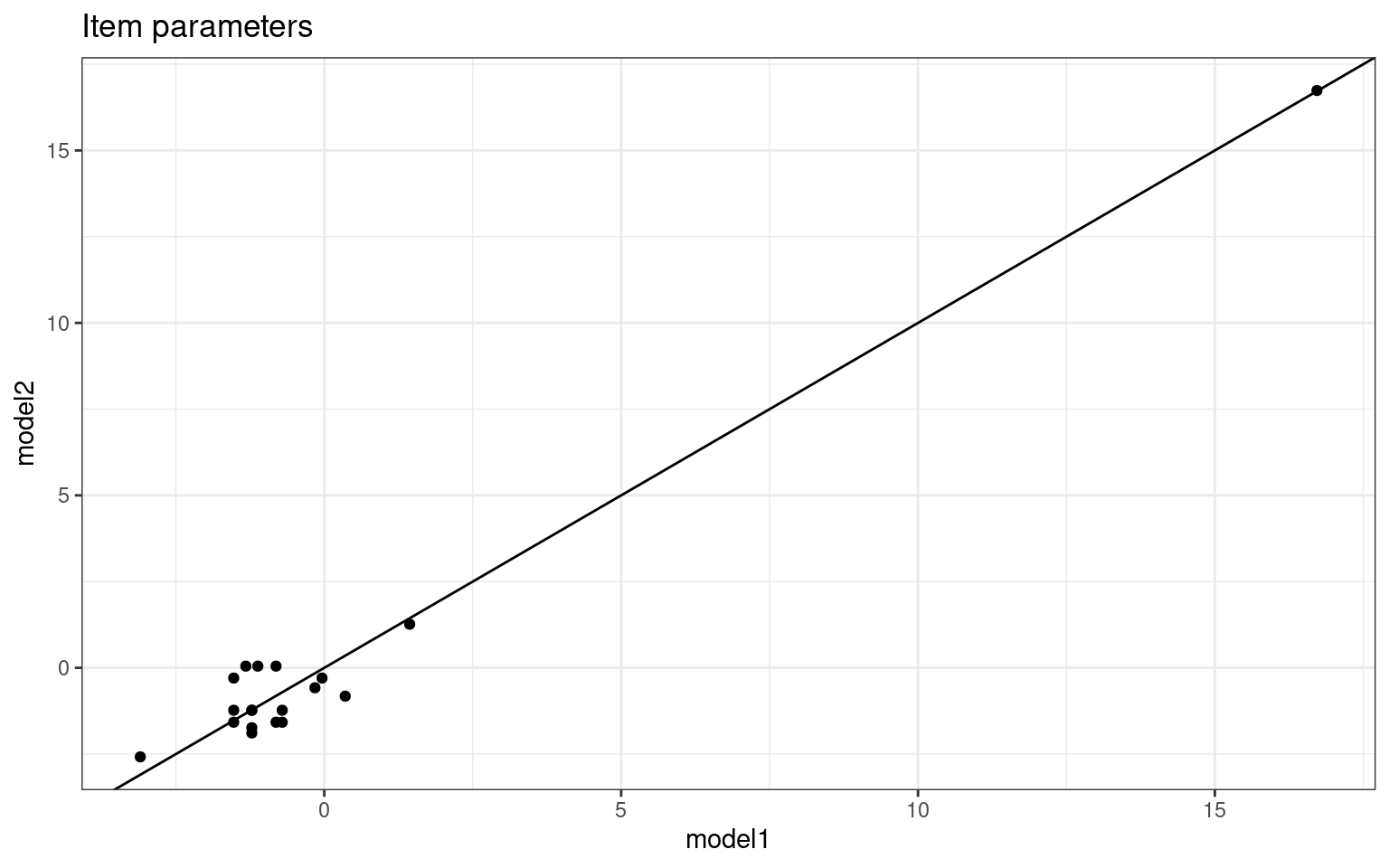cor(model$coef[1:numsubs], skilllevel)
 0.8558655

This is not bad–we predict fairly well the skill level of each person based on 10 yes/no answers. How about assessing the question difficulty:

qplot(x = c(0, (model$coef[-(1:numsubs)])), y = questiondiff) + geom_label(label = 1:length(questiondiff)) + xlab("Estimated Model coefficient") + ylab("Question difficulty")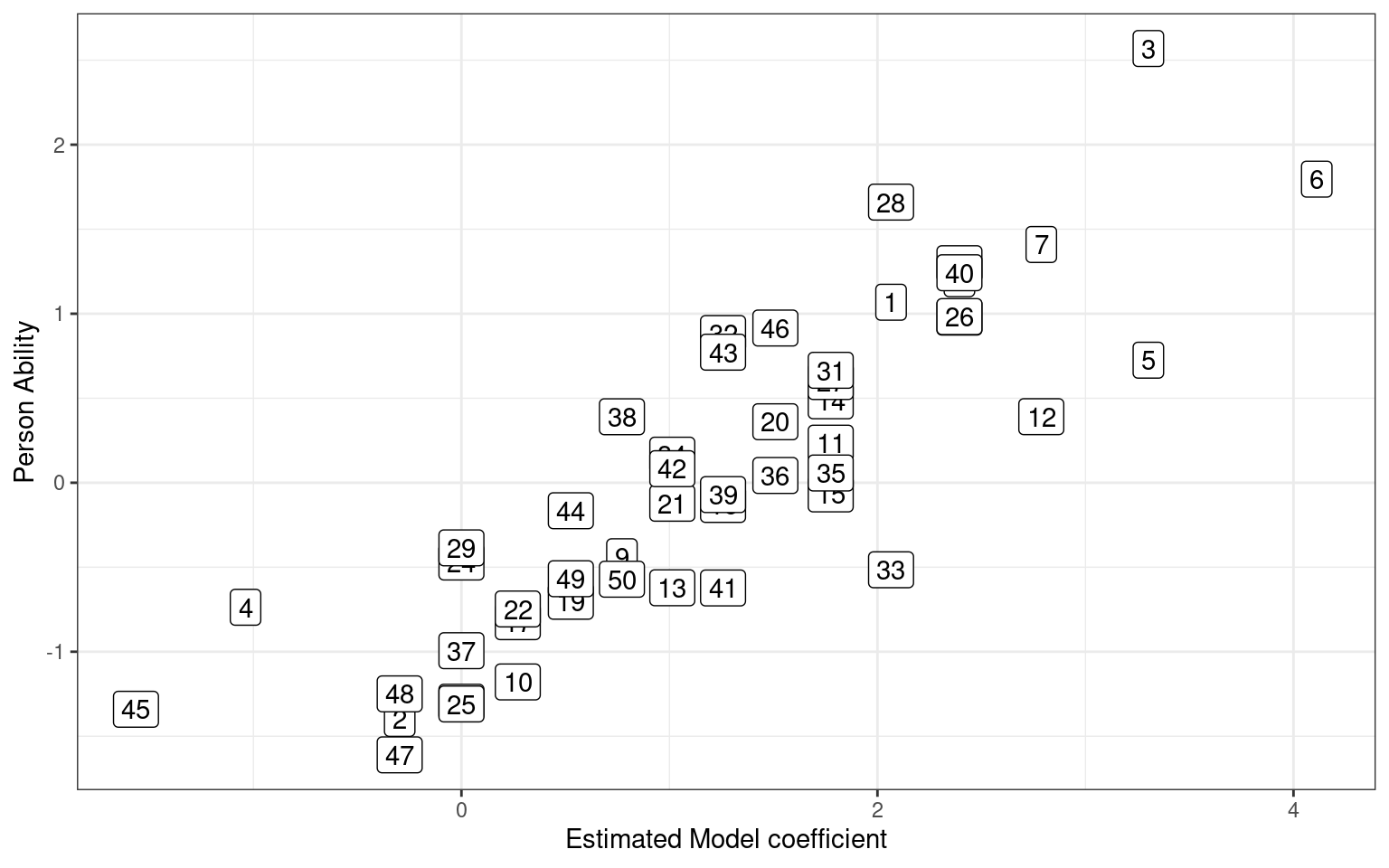cor(c(0, model$coef[-(1:numsubs)]), questiondiff)
 -0.8100234

We could have scored each person and each question according to accuracy:

rowMeans(sim1)
  0.75 0.30 0.90 0.20 0.90 0.95 0.85 0.80 0.50 0.40 0.70 0.85 0.55 0.70
 0.70 0.60 0.40 0.35 0.45 0.65 0.55 0.40 0.80 0.35 0.35 0.80 0.70 0.75
 0.35 0.80 0.70 0.60 0.75 0.55 0.70 0.65 0.35 0.50 0.60 0.80 0.60 0.55
 0.60 0.45 0.15 0.65 0.30 0.30 0.45 0.50
colMeans(sim1)
  0.90 0.50 0.60 1.00 0.58 0.50 0.58 0.44 0.72 0.44 0.52 0.70 0.78 0.60
 0.18 0.50 0.48 0.50 0.44 0.68
par(mfrow = c(1, 2))
plot(rowMeans(sim1), model$coef[1:numsubs], xlab = "Question accuracy", ylab = "Question difficulty parameter") plot(colMeans(sim1), c(0, model$coef[-(1:numsubs)]), xlab = "Person accuracy",
ylab = "Person ability parameter")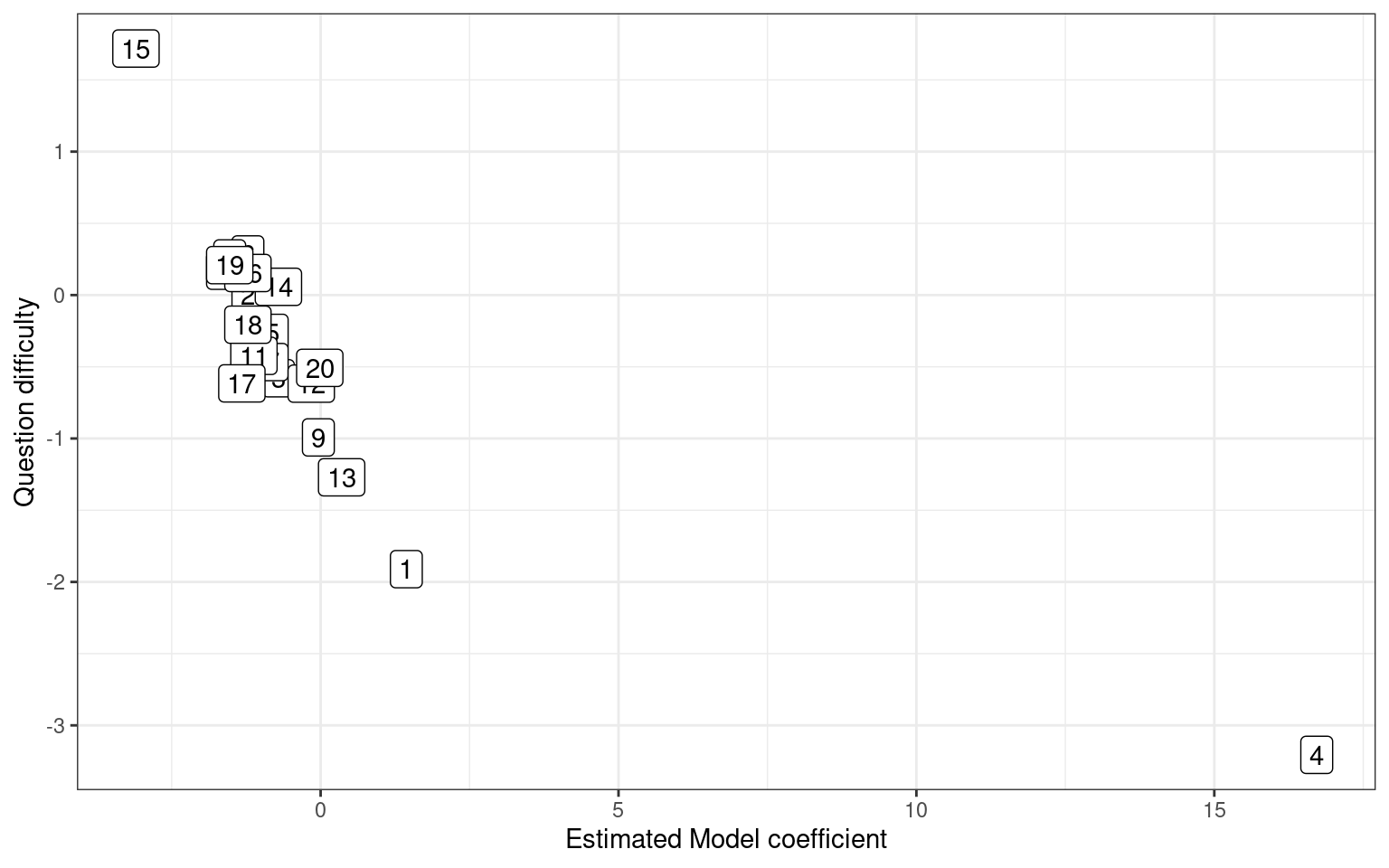par(mfrow = c(1, 2))
plot(rowMeans(sim2), model2$coef[1:numsubs], xlab = "Question accuracy", ylab = "Question difficulty parameter") plot(colMeans(sim2), c(0, model2$coef[-(1:numsubs)]), xlab = "Person accuracy",
ylab = "Person ability parameter")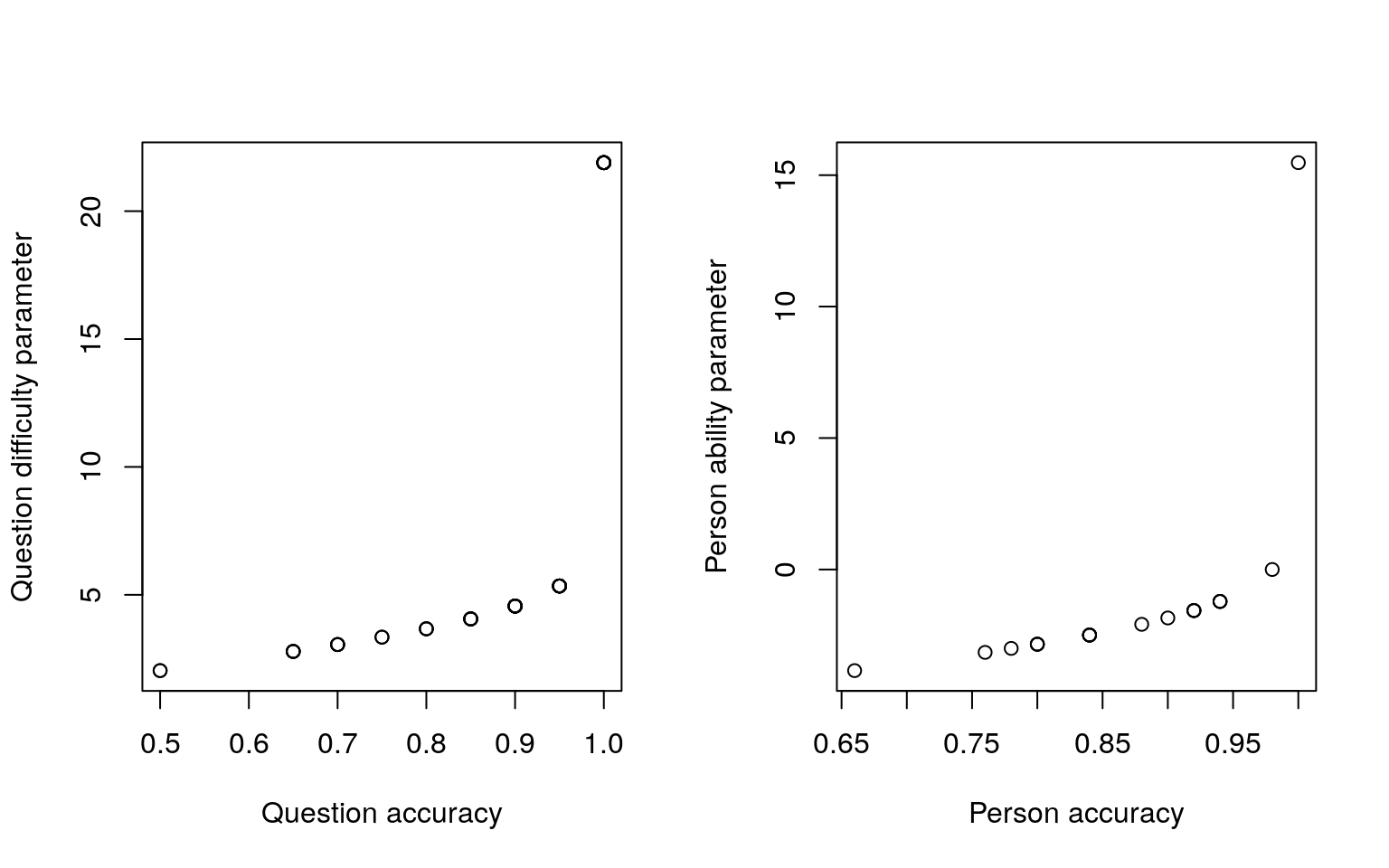Notice that there is a fairly strong mapping between the question accuracy and the difficulty. What if we look at the two different tests and compare parameter estimates:

abilities <- data.frame(set1 = model$coef[1:50], set2 = model2$coef[1:50])
cor(abilities)
          set1      set2
set1 1.0000000 0.5772977
set2 0.5772977 1.0000000
ggplot(abilities, aes(x = set1, y = set2)) + geom_point() + ggtitle("Person abilities")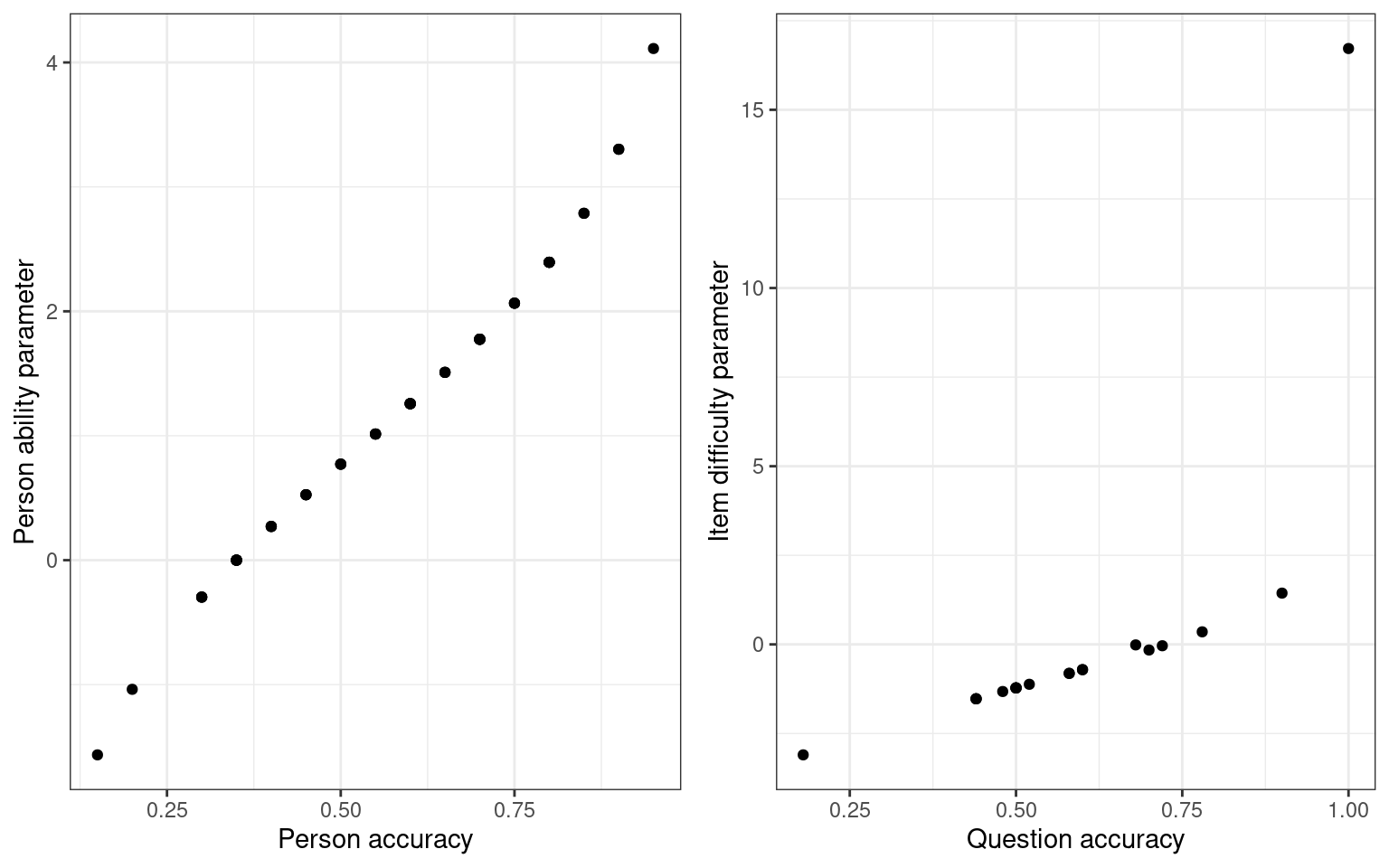probdifficulty <- data.frame(set1 = model$coef[-(1:50)], set2 = model2$coef[-(1:50)])

cor(probdifficulty)
          set1      set2
set1 1.0000000 0.9840795
set2 0.9840795 1.0000000
ggplot(probdifficulty, aes(x = set1, y = set2)) + geom_point() + ggtitle("Question difficulty")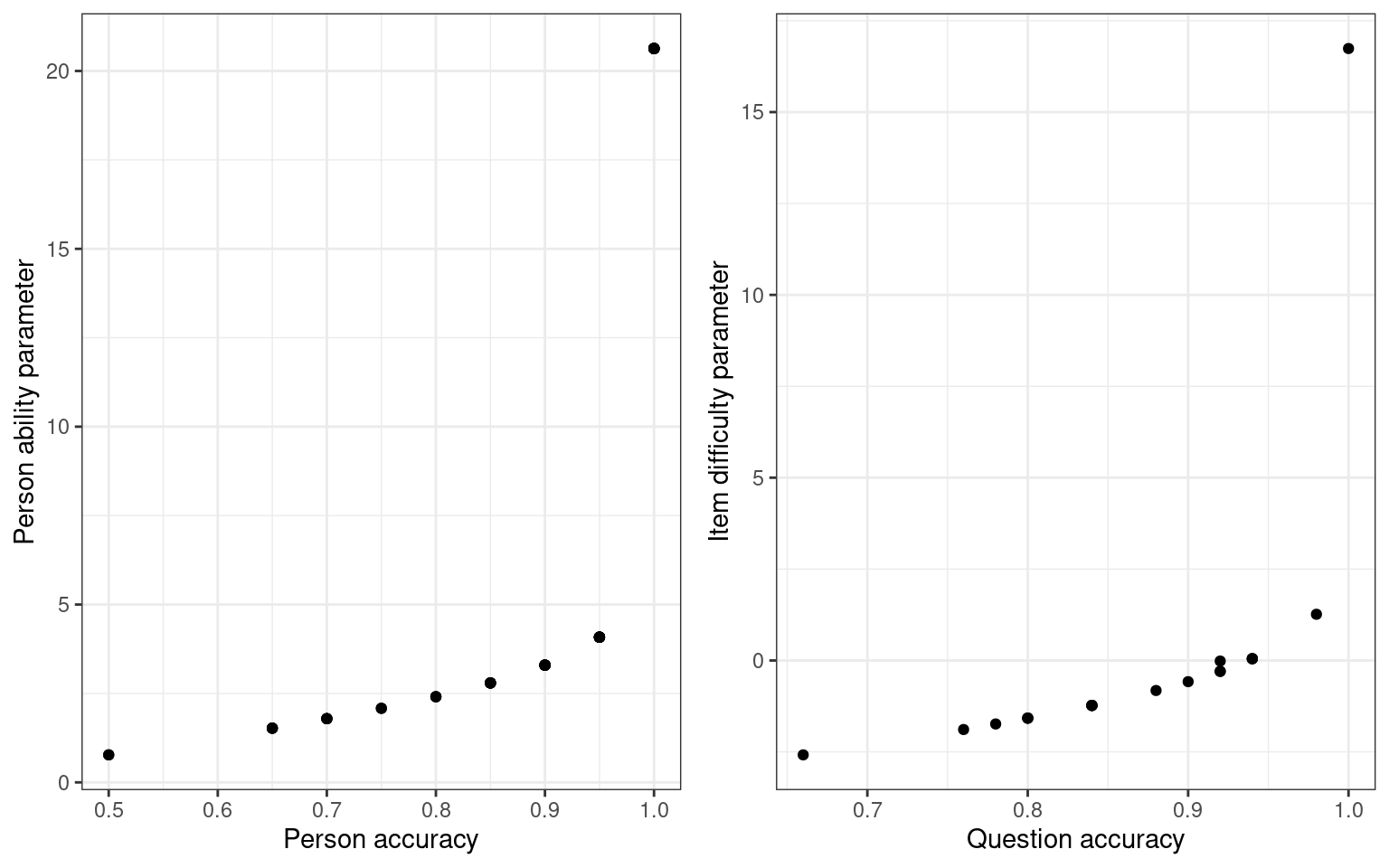We are able to extract ‘person’ related coefficients across the two tests that are reasonably well related. Furthermore, we get high correlations for the test parameters

This analysis is equivalent to what is known as the Rasch model of Item Response Theory (IRT). The ltm package estimates these directly from a wide data format

library(ltm)

p1 <- sim1 + 0
p2 <- sim2 + 0
irt1 <- rasch(p1)
irt2 <- rasch(p2)
plot(irt1)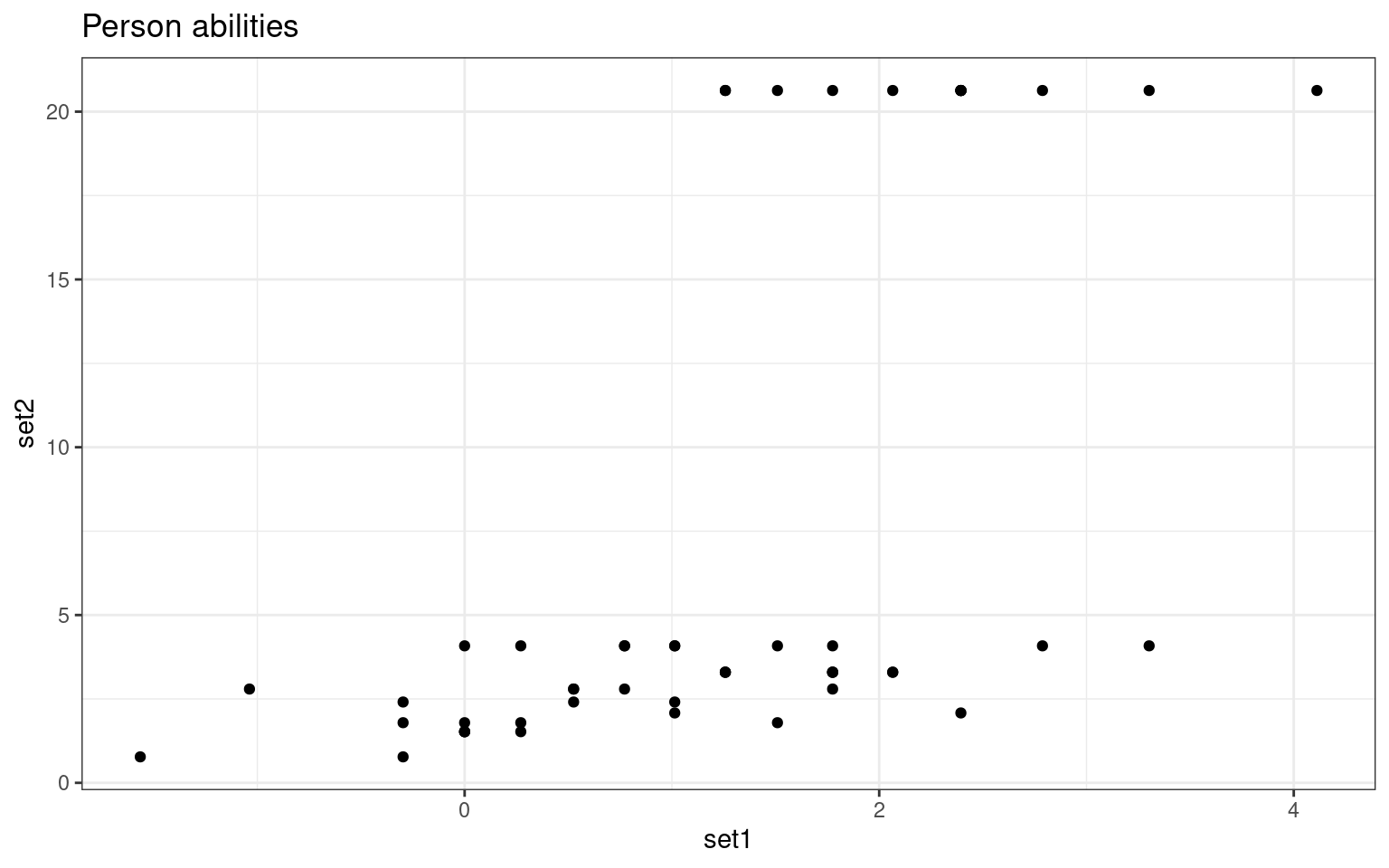plot(irt2)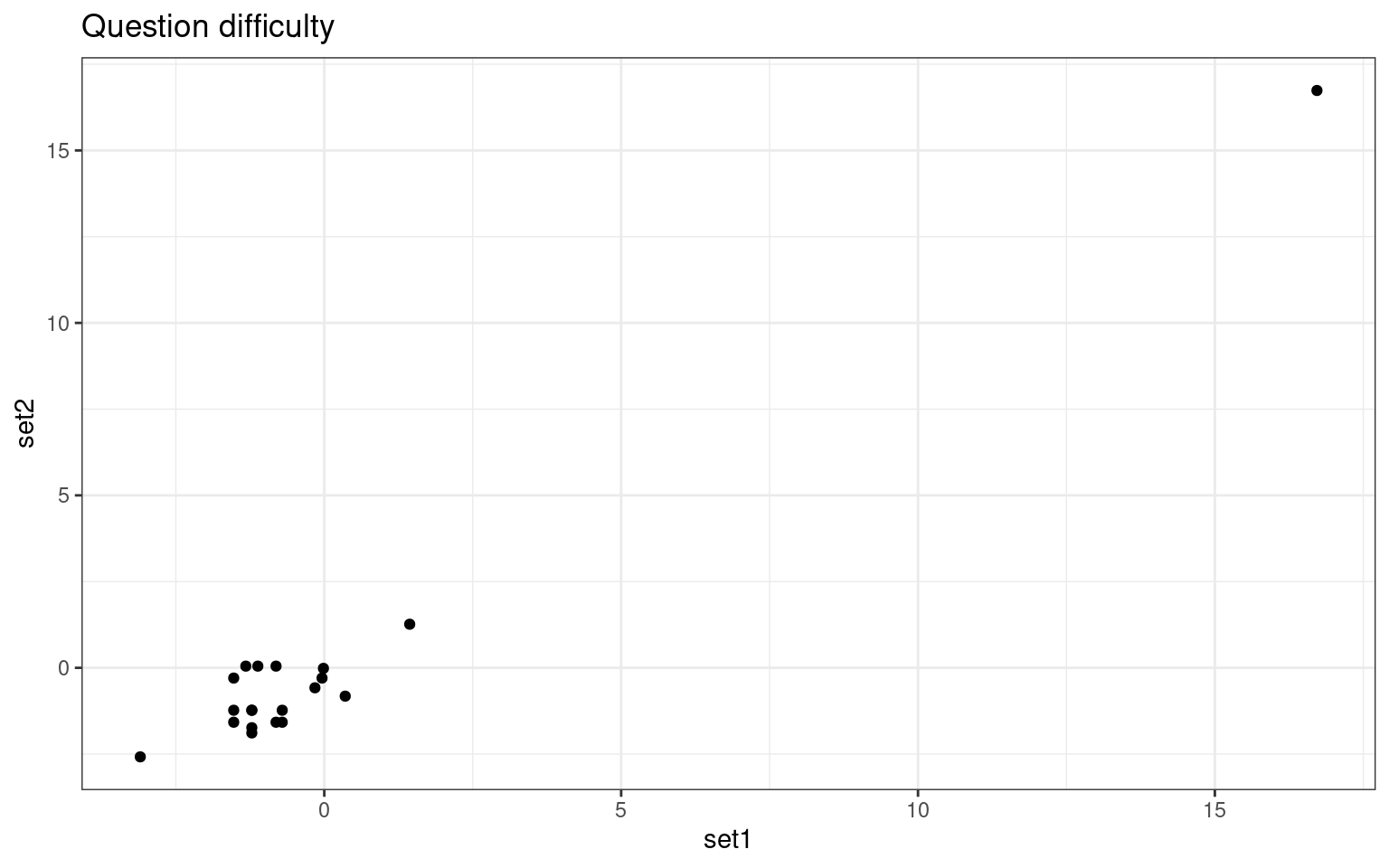summary(irt1)

Call:
rasch(data = p1)

Model Summary:
log.Lik      AIC      BIC
-565.682 1173.364 1213.517

Coefficients:
value    std.err  z.vals
Dffclt.Item 1   -2.6972     0.6433 -4.1927
Dffclt.Item 2    0.0009     0.3591  0.0026
Dffclt.Item 3   -0.5142     0.3703 -1.3888
Dffclt.Item 4  -28.3941 71439.4339 -0.0004
Dffclt.Item 5   -0.4094     0.3662 -1.1180
Dffclt.Item 6    0.0008     0.3591  0.0024
Dffclt.Item 7   -0.4095     0.3662 -1.1181
Dffclt.Item 8    0.3074     0.3630  0.8467
Dffclt.Item 9   -1.1912     0.4173 -2.8544
Dffclt.Item 10   0.3074     0.3630  0.8469
Dffclt.Item 11  -0.1009     0.3595 -0.2806
Dffclt.Item 12  -1.0699     0.4063 -2.6331
Dffclt.Item 13  -1.5870     0.4606 -3.4453
Dffclt.Item 14  -0.5143     0.3703 -1.3890
Dffclt.Item 15   1.8911     0.5009  3.7752
Dffclt.Item 16   0.0010     0.3591  0.0027
Dffclt.Item 17   0.1027     0.3595  0.2857
Dffclt.Item 18   0.0009     0.3591  0.0026
Dffclt.Item 19   0.3074     0.3630  0.8469
Dffclt.Item 20  -0.9533     0.3968 -2.4027
Dscrmn           0.9356     0.1343  6.9684

Integration:
method: Gauss-Hermite

Optimization:
Convergence: 0
quasi-Newton: BFGS 
summary(irt2)

Call:
rasch(data = p2)

Model Summary:
log.Lik      AIC      BIC
-335.3924 712.7847 752.9372

Coefficients:
value    std.err  z.vals
Dffclt.Item 1   -3.7372     1.0006 -3.7349
Dffclt.Item 2   -1.3398     0.3768 -3.5559
Dffclt.Item 3   -1.4609     0.3917 -3.7292
Dffclt.Item 4  -21.8561 48471.2855 -0.0005
Dffclt.Item 5   -1.4608     0.3917 -3.7291
Dffclt.Item 6   -1.2254     0.3639 -3.3676
Dffclt.Item 7   -2.7467     0.6423 -4.2763
Dffclt.Item 8   -2.4679     0.5719 -4.3151
Dffclt.Item 9   -2.4674     0.5718 -4.3151
Dffclt.Item 10  -1.4609     0.3917 -3.7291
Dffclt.Item 11  -2.7455     0.6420 -4.2766
Dffclt.Item 12  -2.2411     0.5219 -4.2938
Dffclt.Item 13  -2.0490     0.4842 -4.2320
Dffclt.Item 14  -1.7281     0.4296 -4.0226
Dffclt.Item 15  -0.7187     0.3212 -2.2373
Dffclt.Item 16  -1.7277     0.4295 -4.0222
Dffclt.Item 17  -2.7448     0.6418 -4.2767
Dffclt.Item 18  -1.7277     0.4295 -4.0223
Dffclt.Item 19  -1.7277     0.4295 -4.0223
Dffclt.Item 20  -2.4680     0.5720 -4.3151
Dscrmn           1.2155     0.2000  6.0774

Integration:
method: Gauss-Hermite

Optimization:
Convergence: 0
quasi-Newton: BFGS 
## this is an alternative to alpha in psych package
descript(p1)

Descriptive statistics for the 'p1' data-set

Sample:
20 items and 50 sample units; 0 missing values

Proportions for each level of response:
[]
 0.1 0.9

[]
 0.5 0.5

[]
 0.4 0.6

[]
 1

[]
 0.42 0.58

[]
 0.5 0.5

[]
 0.42 0.58

[]
 0.56 0.44

[]
 0.28 0.72

[]
 0.56 0.44

[]
 0.48 0.52

[]
 0.3 0.7

[]
 0.22 0.78

[]
 0.4 0.6

[]
 0.82 0.18

[]
 0.5 0.5

[]
 0.52 0.48

[]
 0.5 0.5

[]
 0.56 0.44

[]
 0.32 0.68

Frequencies of total scores:
0 1 2 3 4 5 6 7 8 9 10 11 12 13 14 15 16 17 18 19 20
Freq 0 0 0 1 1 0 3 5 3 3  3  4  5  3  6  3  5  2  2  1  0

Cronbach's alpha:
value
All Items         0.7616
Excluding Item 1  0.7542
Excluding Item 2  0.7527
Excluding Item 3  0.7553
Excluding Item 4  0.7637
Excluding Item 5  0.7543
Excluding Item 6  0.7371
Excluding Item 7  0.7618
Excluding Item 8  0.7559
Excluding Item 9  0.7351
Excluding Item 10 0.7507
Excluding Item 11 0.7338
Excluding Item 12 0.7602
Excluding Item 13 0.7735
Excluding Item 14 0.7457
Excluding Item 15 0.7504
Excluding Item 16 0.7561
Excluding Item 17 0.7468
Excluding Item 18 0.7509
Excluding Item 19 0.7498
Excluding Item 20 0.7484

Pairwise Associations:
Item i Item j p.value
1       1      8   1.000
2       1     12   1.000
3       1     13   1.000
4       1     15   1.000
5       1     18   1.000
6       1     19   1.000
7       2      4   1.000
8       2      7   1.000
9       2     13   1.000
10      2     18   1.000

Compare to our logistic regression:

plot(c(0, model$coef[-(1:50)]), irt1$coef[, 1], main = "Comparison of \nmodel coefficients",
xlab = "Logistic coefficients", ylab = "IRT coefficients")plot(c(0, model2$coef[-(1:50)]), irt2$coef[, 1], main = "Comparison of \nmodel coefficients",
xlab = "Logistic coefficients", ylab = "IRT coefficients")# Visualizing the Rasch Model

If you plot() the model, it will display the inferred logistic curves for all the questions

plot(irt1)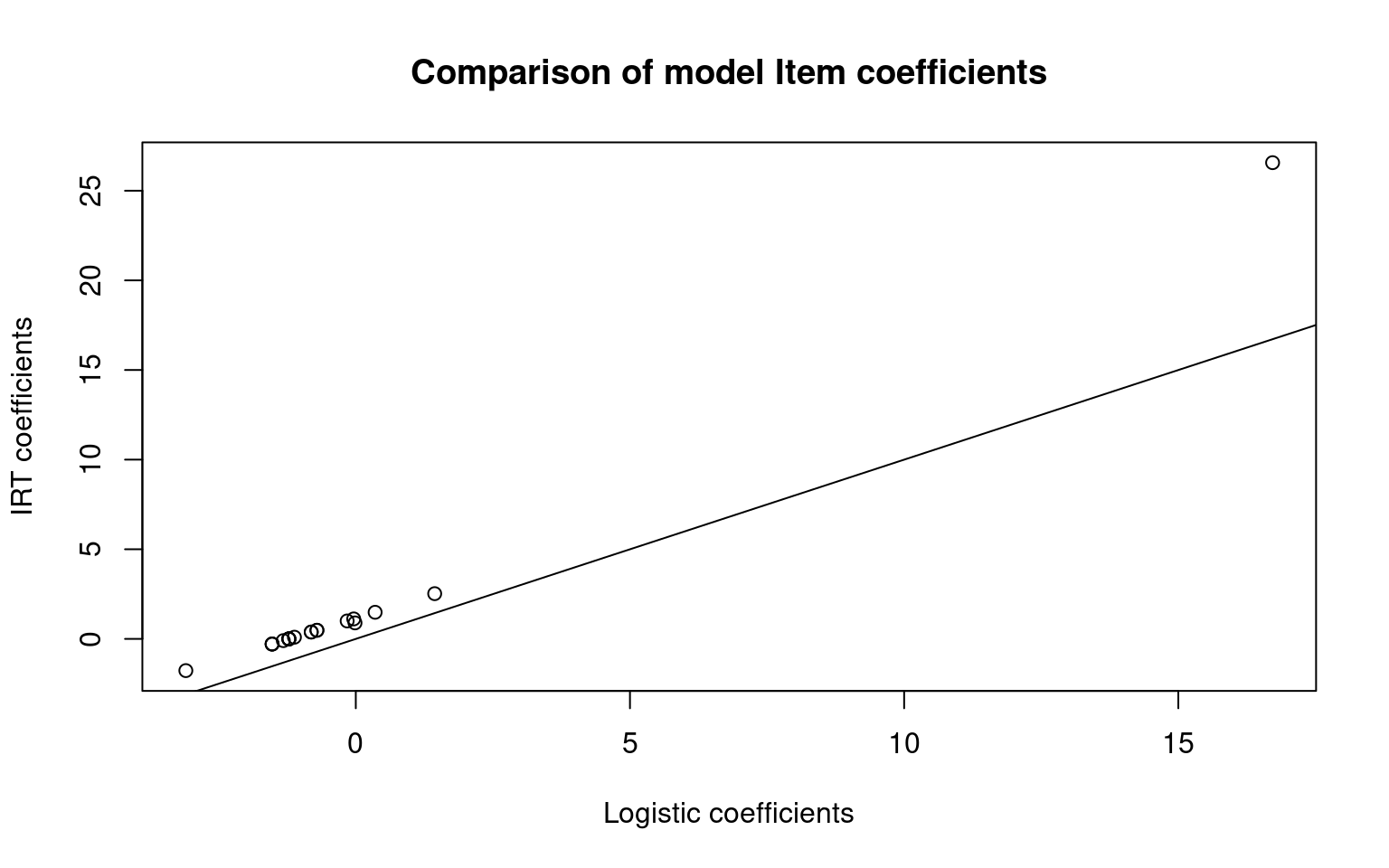plot(irt2)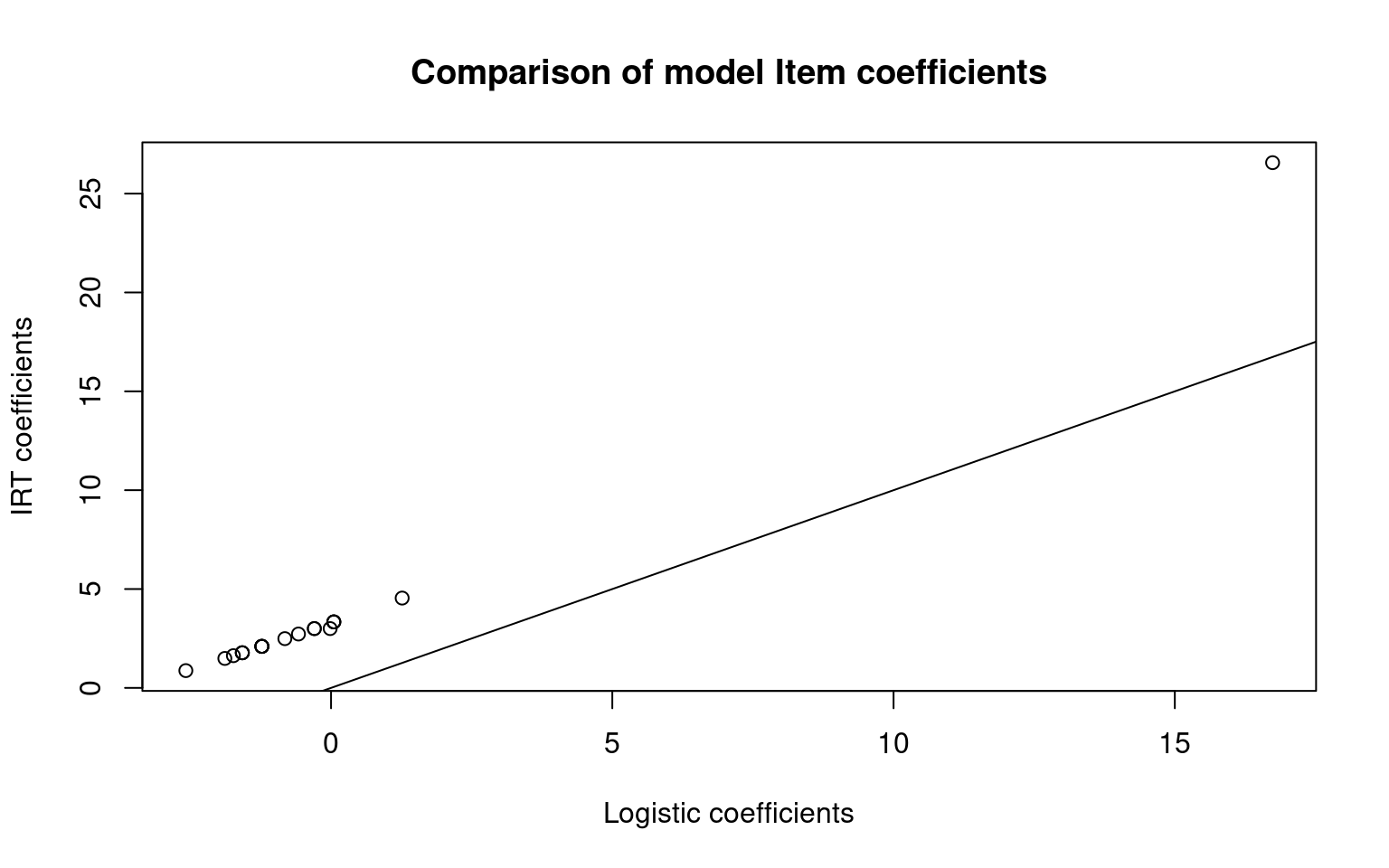Notice that each curve is identical but shifted. The slope of the model is fit as a common value for all items, with different constant offsets (i.e., intercepts) for each question.

## Boundary conditions of the Rasch Model

The data/questions in this example were all created as if they obeyed IRT. Thus, the model worked fairly well. If we have any violations of the model, the estimates can get less precise, and the small number of respondents (50) for the questions we chose (20) would not be enough. Typically you would want more, and the more complicated the model, the more participants.

What happens if they don’t–if we have ‘bad’ questions. One way to do this is to recode a few questions in the opposite direction, so that the people with high ability are more likely to get it wrong

set.seed(10010)
irt2 <- rasch(sim2 + 0)
sim3 <- sim2
sim3[, 1:5] <- (runif(5 * numsubs) < 0.5) + 0
irt3 <- rasch(sim3)
summary(irt2)

Call:
rasch(data = sim2 + 0)

Model Summary:
log.Lik      AIC      BIC
-335.3924 712.7847 752.9372

Coefficients:
value    std.err  z.vals
Dffclt.Item 1   -3.7372     1.0006 -3.7349
Dffclt.Item 2   -1.3398     0.3768 -3.5559
Dffclt.Item 3   -1.4609     0.3917 -3.7292
Dffclt.Item 4  -21.8561 48471.2855 -0.0005
Dffclt.Item 5   -1.4608     0.3917 -3.7291
Dffclt.Item 6   -1.2254     0.3639 -3.3676
Dffclt.Item 7   -2.7467     0.6423 -4.2763
Dffclt.Item 8   -2.4679     0.5719 -4.3151
Dffclt.Item 9   -2.4674     0.5718 -4.3151
Dffclt.Item 10  -1.4609     0.3917 -3.7291
Dffclt.Item 11  -2.7455     0.6420 -4.2766
Dffclt.Item 12  -2.2411     0.5219 -4.2938
Dffclt.Item 13  -2.0490     0.4842 -4.2320
Dffclt.Item 14  -1.7281     0.4296 -4.0226
Dffclt.Item 15  -0.7187     0.3212 -2.2373
Dffclt.Item 16  -1.7277     0.4295 -4.0222
Dffclt.Item 17  -2.7448     0.6418 -4.2767
Dffclt.Item 18  -1.7277     0.4295 -4.0223
Dffclt.Item 19  -1.7277     0.4295 -4.0223
Dffclt.Item 20  -2.4680     0.5720 -4.3151
Dscrmn           1.2155     0.2000  6.0774

Integration:
method: Gauss-Hermite

Optimization:
Convergence: 0
quasi-Newton: BFGS 
summary(irt3)

Call:
rasch(data = sim3)

Model Summary:
log.Lik      AIC      BIC
-444.2396 930.4791 970.6316

Coefficients:
value std.err  z.vals
Dffclt.Item 1  -0.2581  0.4654 -0.5546
Dffclt.Item 2   0.3966  0.4693  0.8451
Dffclt.Item 3  -0.5225  0.4755 -1.0989
Dffclt.Item 4   0.2659  0.4652  0.5716
Dffclt.Item 5   0.3968  0.4693  0.8455
Dffclt.Item 6  -1.8679  0.6167 -3.0287
Dffclt.Item 7  -4.3637  1.1820 -3.6919
Dffclt.Item 8  -3.8917  1.0450 -3.7240
Dffclt.Item 9  -3.8921  1.0451 -3.7240
Dffclt.Item 10 -2.2409  0.6778 -3.3061
Dffclt.Item 11 -4.3638  1.1820 -3.6919
Dffclt.Item 12 -3.5111  0.9459 -3.7121
Dffclt.Item 13 -3.1954  0.8705 -3.6707
Dffclt.Item 14 -2.6720  0.7584 -3.5231
Dffclt.Item 15 -1.0778  0.5170 -2.0847
Dffclt.Item 16 -2.6712  0.7583 -3.5228
Dffclt.Item 17 -4.3641  1.1821 -3.6918
Dffclt.Item 18 -2.6713  0.7583 -3.5228
Dffclt.Item 19 -2.6720  0.7584 -3.5231
Dffclt.Item 20 -3.8914  1.0450 -3.7240
Dscrmn          0.6761  0.1298  5.2080

Integration:
method: Gauss-Hermite

Optimization:
Convergence: 0
quasi-Newton: BFGS 
plot((cbind(irt1$coef[, 1], irt3$coef[, 1])), main = "Item coefficients with bad questions",
xlab = "test 2", ylab = "test 3")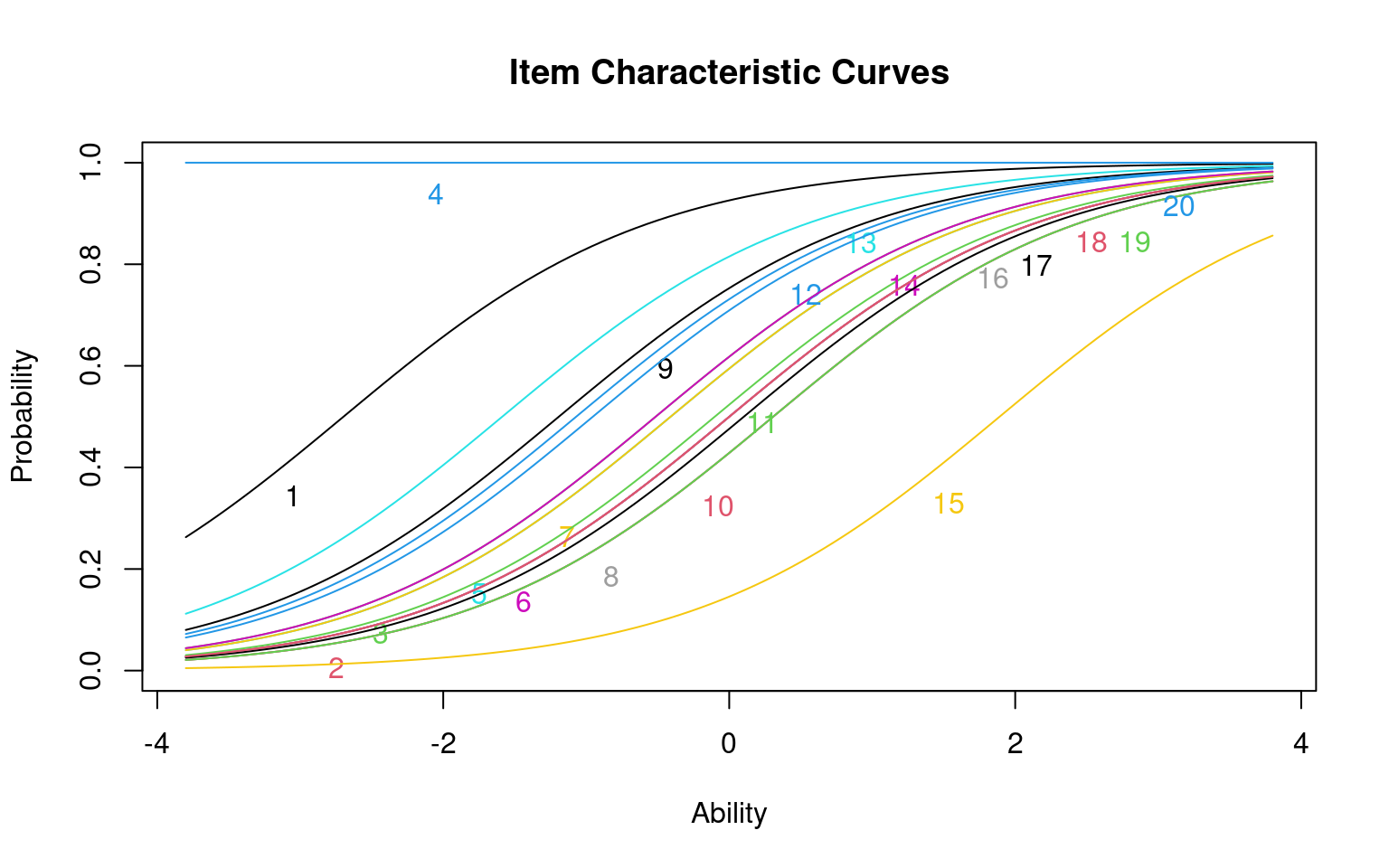plot((cbind(irt1$coef[, 1], irt3$coef[, 1])), main = "Item coefficients with bad questions (zoomed)",
xlab = "test 2", ylab = "test 3", ylim = c(-5, 5))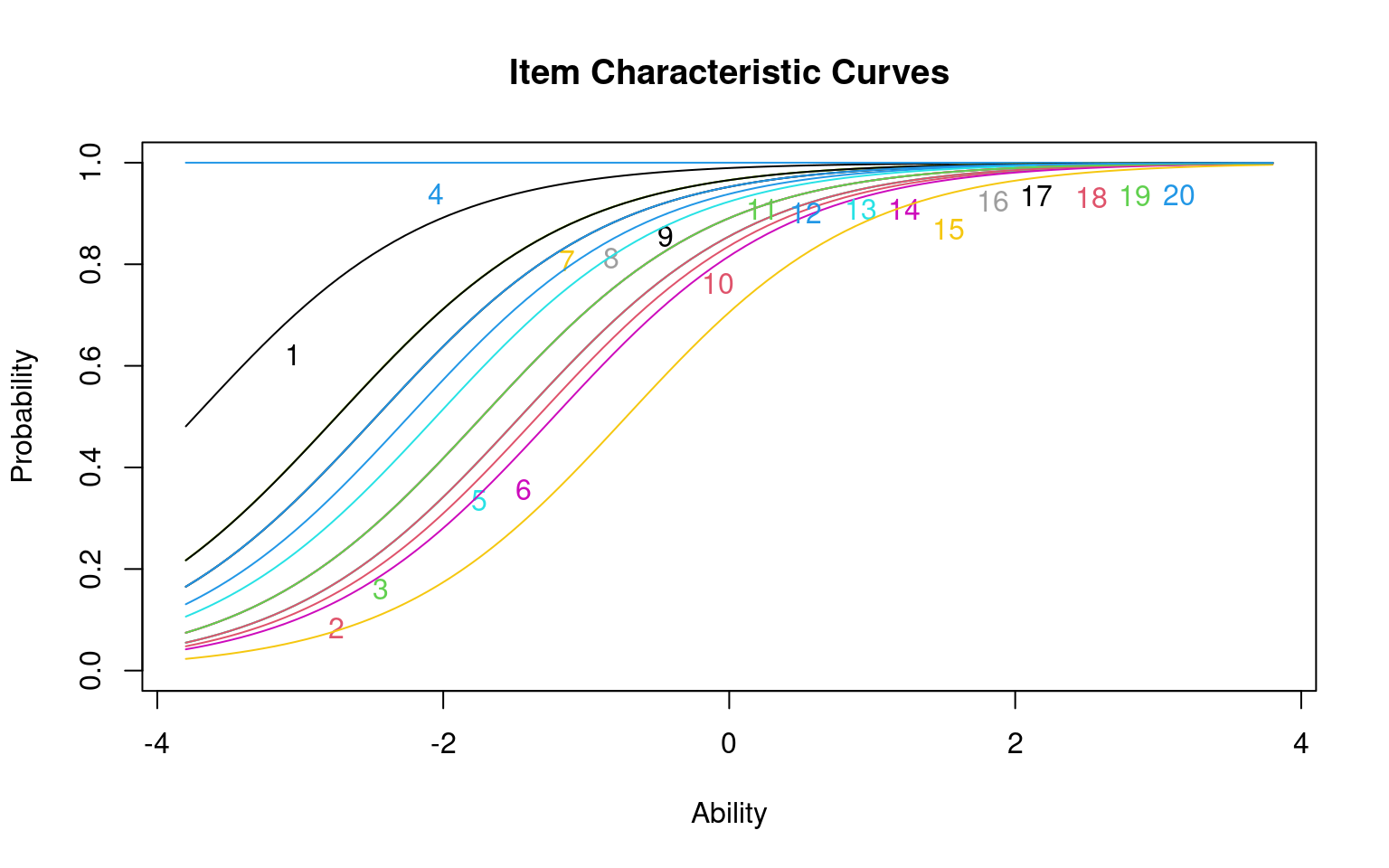In this case, the ‘bad’ questions all ended up with negative difficulty coefficients. If we examine the questions using item.fit, it will test whether each question fits into the basic model. When everything was from the model, none of the items were selected as bad. Once we made 5 items (25%) bad, in this case a bunch of items get flagged. This includes all the items 1..5, but also 6, 7, and 9 and maybe 18. Strangely, a few bad questions might make other questions look bad as well.

item.fit(irt2)

Item-Fit Statistics and P-values

Call:
rasch(data = sim2 + 0)

Alternative: Items do not fit the model
Ability Categories: 10

X^2 Pr(>X^2)
It 1   2.4798   0.9286
It 2  13.5244   0.0603
It 3   5.2193   0.6332
It 4   0.0000        1
It 5  13.3812   0.0633
It 6   6.6327   0.4681
It 7   3.6856   0.8152
It 8   4.6883   0.6979
It 9  14.4979    0.043
It 10  8.9407   0.2569
It 11  6.8692   0.4426
It 12  5.4804   0.6016
It 13 10.4973   0.1621
It 14  4.3378   0.7401
It 15  5.0282   0.6565
It 16  8.0741   0.3261
It 17  7.3144   0.3969
It 18  7.9045   0.3411
It 19  9.9131   0.1936
It 20  4.0405   0.7751
item.fit(irt3)

Item-Fit Statistics and P-values

Call:
rasch(data = sim3)

Alternative: Items do not fit the model
Ability Categories: 10

X^2 Pr(>X^2)
It 1   3.5064   0.7431
It 2   3.8323   0.6994
It 3   2.5409   0.8639
It 4   5.8604    0.439
It 5   8.6429   0.1947
It 6   3.9761   0.6799
It 7   4.3075   0.6351
It 8   7.5153   0.2758
It 9   3.8459   0.6975
It 10  5.2996    0.506
It 11  3.0357   0.8044
It 12  7.8566   0.2488
It 13  3.3830   0.7595
It 14  6.1092   0.4111
It 15 10.6329   0.1004
It 16  3.8659   0.6948
It 17 10.7470   0.0965
It 18  2.6317   0.8534
It 19  3.6177   0.7283
It 20  2.8663   0.8254

Some of the other things we can look at to examine the fit of the model:

person.fit(irt2)

Person-Fit Statistics and P-values

Call:
rasch(data = sim2 + 0)

Alternative: Inconsistent response pattern under the estimated model

It 1 It 2 It 3 It 4 It 5 It 6 It 7 It 8 It 9 It 10 It 11 It 12 It 13
1     0    0    0    1    0    1    1    0    1     1     1     1     1
2     1    0    0    1    0    0    0    1    1     1     1     0     0
3     1    0    0    1    0    0    1    1    1     0     0     0     1
It 14 It 15 It 16 It 17 It 18 It 19 It 20       L0      Lz Pr(<Lz)
1      1     0     1     1     0     1     1 -12.6539 -1.0533  0.1461
2      0     0     1     1     0     1     1 -10.8261  0.6039  0.7271
3      0     0     0     1     1     1     1 -10.1098  1.0793  0.8598
[ reached getOption("max.print") -- omitted 33 rows ]
person.fit(irt3)

Person-Fit Statistics and P-values

Call:
rasch(data = sim3)

Alternative: Inconsistent response pattern under the estimated model

It 1 It 2 It 3 It 4 It 5 It 6 It 7 It 8 It 9 It 10 It 11 It 12 It 13
1     0    0    0    0    1    1    1    1    1     0     1     0     1
2     0    0    0    0    1    1    1    1    1     0     1     1     1
3     0    0    0    1    0    0    0    1    1     1     1     0     0
It 14 It 15 It 16 It 17 It 18 It 19 It 20       L0      Lz Pr(<Lz)
1      0     1     0     1     1     0     1 -12.1349 -0.6812  0.2479
2      1     0     0     0     1     1     1 -10.9041 -0.2671  0.3947
3      0     0     1     1     0     1     1 -12.9750 -0.8758  0.1906
[ reached getOption("max.print") -- omitted 43 rows ]

# Extending and Constraining IRT

## Slope of the item characteristic function

In the Rasch model, all items are of the same family, and have the same slope, or steepness. A very steep function means that there is a sharp cut-off between who can answer it correctly and who cannot. This is often called ‘discriminability’. A good test item typically has high discriminabilty, and a good test has a set of highly-discriminable items that have different difficulty. Typically, high discriminability will correspond to good part-whole item correlations. As a sort of ideal situation, the easiest item will be answered correctly by everyone but the lowest-ability person, the hardest item will only be answered correctly by the highest-ability person, and the person’s ability will directly control how many of the items they can answer. As a rule of thumb, higher discriminability values (greater than 1.0, or better yet greater than 2.0) are good. By default, the rasch model estimates a slope. However, the default logistic model will have a slope of 1.0, and so this is sometimes considered a simpler model. You might do this if you have limited data–maybe a test from a class with relatively few students, because it will hopefully make estimation more stable.

For example, The following is are the results of a psychology test:

dat <- read.csv("testscores.csv")

## descript(dat) ##doesn't work. Thus compute Cronbach's alahp on the data
descript(dat, chi.squared = F)

Descriptive statistics for the 'dat' data-set

Sample:
47 items and 21 sample units; 0 missing values

Proportions for each level of response:
$q1 1 1$q2
0      1
0.3333 0.6667

$q3 1 1$q4
0      1
0.8095 0.1905

$q5 0 1 0.1429 0.8571$q6
0      1
0.3333 0.6667

$q7 0 1 0.2381 0.7619$q8
0      1
0.1429 0.8571

$q9 1 1$q10
0      1
0.0476 0.9524

$q11 0 1 0.619 0.381$q12
0     1
0.381 0.619

$q13 0 1 0.0476 0.9524$q14
1
1

$q15 0 1 0.7619 0.2381$q16
0      1
0.0476 0.9524

$q17 1 1$q18
0      1
0.0476 0.9524

$q19 0 1 0.3333 0.6667$q20
0      1
0.2381 0.7619

$q21 1 1$q22
0      1
0.2857 0.7143

$q23 0 1 0.2381 0.7619$q24
1
1

$q25 0 1 0.6667 0.3333$q26
1
1

$q27 0 1 0.7143 0.2857$q28
0     1
0.381 0.619

$q29 0 1 0.381 0.619$q31
0      1
0.6667 0.3333

$q32 0 1 0.6667 0.3333$q33
0      1
0.4762 0.5238

$q34 0 1 0.0952 0.9048$q35
0      1
0.3333 0.6667

$q36 0 1 0.619 0.381$q37
0      1
0.3333 0.6667

$q38 0 1 0.1905 0.8095$q39
0      1
0.7619 0.2381

$q40 0 1 0.4762 0.5238$q41
0      1
0.0476 0.9524

$q42 0 1 0.1429 0.8571$q43
0      1
0.0952 0.9048

$q44 0 1 0.381 0.619$q45
0     1
0.381 0.619

$q47 0 1 0.2381 0.7619$q48
0     1
0.619 0.381

$q49 0 1 0.7143 0.2857 Frequencies of total scores: 0 1 2 3 4 5 6 7 8 9 10 11 12 13 14 15 16 17 18 19 20 21 22 23 24 25 Freq 0 0 0 0 0 0 0 0 0 0 0 0 0 0 0 0 0 0 0 0 0 0 0 0 0 0 26 27 28 29 30 31 32 33 34 35 36 37 38 39 40 41 42 43 44 45 46 47 Freq 0 3 0 2 3 3 2 1 1 1 0 0 1 3 0 1 0 0 0 0 0 0 Cronbach's alpha: value All Items 0.6291 Excluding q1 0.6294 Excluding q2 0.6504 Excluding q3 0.6294 Excluding q4 0.6317 Excluding q5 0.6138 Excluding q6 0.5851 Excluding q7 0.6021 Excluding q8 0.6313 Excluding q9 0.6294 Excluding q10 0.6211 Excluding q11 0.6217 Excluding q12 0.6122 Excluding q13 0.6297 Excluding q14 0.6294 Excluding q15 0.6309 Excluding q16 0.6254 Excluding q17 0.6294 Excluding q18 0.6297 Excluding q19 0.5979 Excluding q20 0.6139 Excluding q21 0.6294 Excluding q22 0.6342 Excluding q23 0.6251 Excluding q24 0.6294 Excluding q25 0.6426 Excluding q26 0.6294 Excluding q27 0.6118 Excluding q28 0.5952 Excluding q29 0.6168 Excluding q31 0.6124 Excluding q32 0.6147 Excluding q33 0.6177 Excluding q34 0.6232 Excluding q35 0.6302 Excluding q36 0.6526 Excluding q37 0.6406 Excluding q38 0.6174 Excluding q39 0.6200 Excluding q40 0.6107 Excluding q41 0.6317 Excluding q42 0.6334 Excluding q43 0.6337 Excluding q44 0.6075 Excluding q45 0.6425 Excluding q47 0.6116 Excluding q48 0.6428 Excluding q49 0.6047 # force the discrimination praameter to be 1 model1 <- rasch(dat, constraint = cbind(length(dat) + 1, 1)) model1  Call: rasch(data = dat, constraint = cbind(length(dat) + 1, 1)) Coefficients: Dffclt.q1 Dffclt.q2 Dffclt.q3 Dffclt.q4 Dffclt.q5 Dffclt.q6 -25.566 -0.773 -25.566 1.591 -1.947 -0.773 Dffclt.q7 Dffclt.q8 Dffclt.q9 Dffclt.q10 Dffclt.q11 Dffclt.q12 -1.281 -1.946 -25.566 -3.188 0.531 -0.546 Dffclt.q13 Dffclt.q14 Dffclt.q15 Dffclt.q16 Dffclt.q17 Dffclt.q18 -3.188 -25.566 1.281 -3.188 -25.566 -3.188 Dffclt.q19 Dffclt.q20 Dffclt.q21 Dffclt.q22 Dffclt.q23 Dffclt.q24 -0.773 -1.281 -25.566 -1.016 -1.281 -25.566 Dffclt.q25 Dffclt.q26 Dffclt.q27 Dffclt.q28 Dffclt.q29 Dffclt.q31 0.762 -25.566 1.009 -0.545 -0.546 0.762 Dffclt.q32 Dffclt.q33 Dffclt.q34 Dffclt.q35 Dffclt.q36 Dffclt.q37 0.762 -0.114 -2.425 -0.773 0.531 -0.773 Dffclt.q38 Dffclt.q39 Dffclt.q40 Dffclt.q41 Dffclt.q42 Dffclt.q43 -1.583 1.281 -0.115 -3.188 -1.946 -2.424 Dffclt.q44 Dffclt.q45 Dffclt.q47 Dffclt.q48 Dffclt.q49 Dscrmn -0.546 -0.545 -1.281 0.531 1.009 1.000 Log.Lik: -430.349 # summary(model1) allow discrimination parameter to be estimated model2 <- rasch(dat) model2  Call: rasch(data = dat) Coefficients: Dffclt.q1 Dffclt.q2 Dffclt.q3 Dffclt.q4 Dffclt.q5 Dffclt.q6 -49.028 -1.416 -49.028 2.930 -3.615 -1.418 Dffclt.q7 Dffclt.q8 Dffclt.q9 Dffclt.q10 Dffclt.q11 Dffclt.q12 -2.362 -3.612 -49.028 -5.965 0.984 -0.996 Dffclt.q13 Dffclt.q14 Dffclt.q15 Dffclt.q16 Dffclt.q17 Dffclt.q18 -5.966 -49.028 2.361 -5.967 -49.028 -5.966 Dffclt.q19 Dffclt.q20 Dffclt.q21 Dffclt.q22 Dffclt.q23 Dffclt.q24 -1.417 -2.364 -49.028 -1.869 -2.362 -49.028 Dffclt.q25 Dffclt.q26 Dffclt.q27 Dffclt.q28 Dffclt.q29 Dffclt.q31 1.407 -49.028 1.862 -0.996 -0.996 1.408 Dffclt.q32 Dffclt.q33 Dffclt.q34 Dffclt.q35 Dffclt.q36 Dffclt.q37 1.408 -0.206 -4.517 -1.416 0.984 -1.416 Dffclt.q38 Dffclt.q39 Dffclt.q40 Dffclt.q41 Dffclt.q42 Dffclt.q43 -2.928 2.361 -0.201 -5.966 -3.612 -4.518 Dffclt.q44 Dffclt.q45 Dffclt.q47 Dffclt.q48 Dffclt.q49 Dscrmn -0.996 -0.996 -2.364 0.984 1.862 0.521 Log.Lik: -426.767 # summary(model2) par(mfrow = c(1, 2)) plot(model1) plot(model2)Notice that several of the questions have difficulty parameters of -49.02. These are the problems that everybody got correct. This also likely led to the error messages returned by the models. It is difficult to estimate the difficulty of such questions, because they must be really easy. We fit two different models; one in which has a discrimination parameter. Is it worthwhile using this extra parameter? anova(model1, model2)  Likelihood Ratio Table AIC BIC log.Lik LRT df p.value model1 954.70 1003.79 -430.35 model2 949.53 999.67 -426.77 7.16 1 0.007 Results show that there is no difference between the two, despite the fact that the mean discriminability when estimated was .5 instead of 1. ## Fitting individual difficulty parameters In other cases, it is likely that different items have different discriminabilities, and you might want to use this to help create a better simpler test. You might be able to choose 5 highly discriminable items to replace 50 low-discriminible items, for example. The two-parameter IRT model can estimate a difficulty and discriminibility for each item. It is fit with the ltm() function in ltm. The ltm function has more bells and whistles that we won’t deal with. For example, it lets you estimate a set of latent predictors–sort of a factor analysis. We will use a single factor, which ends up being the two-parametrer model. The syntax is a bit different. You need to write a formula, and tell it how many latent factors to estimate. We will specify a single factor by doing data~z1, but you can use two by doing data~z1 + z2 model3 <- ltm(dat ~ z1) model3  Call: ltm(formula = dat ~ z1) Coefficients: Dffclt Dscrmn q1 -2.417364e+08 0.000 q2 -7.760000e-01 0.976 q3 -2.417364e+08 0.000 q4 -2.119000e+00 -0.734 q5 1.382000e+00 -22.068 q6 6.560000e-01 -31.827 q7 1.803000e+00 -0.800 q8 1.352400e+01 -0.134 q9 -2.417364e+08 0.000 q10 3.708000e+00 -0.976 q11 -2.900000e-01 -4.347 q12 -3.407000e+00 0.139 q13 -3.773000e+00 0.874 q14 -2.417364e+08 0.000 q15 2.212000e+00 0.608 q16 2.059000e+00 -30.676 q17 -2.417364e+08 0.000 q18 -1.668000e+00 21.067 q19 2.454000e+00 -0.304 q20 2.715000e+00 -0.474 q21 -2.417364e+08 0.000 q22 -1.178080e+02 0.008 q23 -3.029000e+00 0.385 q24 -2.417364e+08 0.000 q25 -5.009000e+00 -0.136 q26 -2.417364e+08 0.000 q27 -1.051000e+00 -0.980 q28 -8.553000e+00 0.056 q29 -2.193000e+00 0.214 q31 -1.614000e+00 -0.423 q32 -2.699000e+00 -0.251 q33 3.370000e-01 -0.445 q34 1.691000e+00 -42.311 q35 4.430000e+00 -0.162 q36 1.202000e+00 0.474 q37 -2.966000e+00 0.229 q38 2.450000e+00 -0.693 [ reached getOption("max.print") -- omitted 10 rows ] Log.Lik: -400.606 plot(model3)# summary(model3) Notice that items vary in their difficulty and discriminibility, and that some are negatively discrimination. It is sort of a mess. This is not unexpected because we have so few participants in this test–there just isn’t enough information to reliably and stably estimate anything. Before we go on, we can look at a few things about how well the model fits: item.fit(model3)  Item-Fit Statistics and P-values Call: ltm(formula = dat ~ z1) Alternative: Items do not fit the model Ability Categories: 10 X^2 Pr(>X^2) q1 0.0000 1 q2 8.1511 0.4189 q3 0.0000 1 q4 7.6834 0.465 q5 0.1379 1 q6 0.2621 1 q7 9.2744 0.3197 q8 8.7169 0.3667 q9 0.0000 1 q10 4.8496 0.7735 q11 1.5435 0.992 q12 3.4025 0.9066 q13 6.8417 0.5538 q14 0.0000 1 q15 6.0480 0.6419 q16 9.9338 0.2697 q17 0.0000 1 q18 133.4230 <0.0001 q19 7.9250 0.4408 q20 6.6433 0.5756 q21 0.0000 1 q22 7.9145 0.4419 q23 11.6092 0.1695 q24 0.0000 1 q25 14.2920 0.0745 q26 0.0000 1 q27 5.5064 0.7023 q28 9.7243 0.2849 q29 7.9233 0.441 q31 6.2791 0.616 q32 6.0579 0.6407 q33 6.5497 0.5859 q34 0.0000 1 q35 9.1083 0.3332 q36 2.4719 0.963 q37 8.9905 0.3431 q38 7.7650 0.4568 [ reached getOption("max.print") -- omitted 10 rows ] This gives a ‘fit’ parameter for each question. A few items, like Q18, have bad fit parameters. Looking at the psych::alpha function, it has very low item-whole correlation. psych::alpha(dat) Some items ( q2 q7 q8 q12 q15 q18 q23 q28 q29 q36 q37 q42 q44 q45 ) were negatively correlated with the total scale and probably should be reversed. To do this, run the function again with the 'check.keys=TRUE' option  Reliability analysis Call: psych::alpha(x = dat) raw_alpha std.alpha G6(smc) average_r S/N ase mean sd median_r 0.63 0.62 1 0.04 1.6 0.11 0.63 0.11 0.038 lower alpha upper 95% confidence boundaries 0.41 0.63 0.85 Reliability if an item is dropped: raw_alpha std.alpha G6(smc) average_r S/N var.r med.r q2 0.65 0.64 1 0.045 1.8 0.050 0.040 q4 0.63 0.62 1 0.042 1.7 0.050 0.037 q5 0.62 0.60 1 0.038 1.5 0.049 0.038 q6 0.59 0.58 1 0.035 1.4 0.049 0.037 q7 0.60 0.60 1 0.037 1.5 0.049 0.037 q8 0.63 0.63 1 0.042 1.7 0.050 0.040 q10 0.62 0.61 1 0.039 1.6 0.050 0.038 q11 0.62 0.61 1 0.040 1.6 0.049 0.038 q12 0.62 0.60 1 0.039 1.5 0.050 0.038 q13 0.63 0.62 1 0.042 1.6 0.049 0.040 [ reached getOption("max.print") -- omitted 29 rows ] Item statistics n raw.r std.r r.cor r.drop mean sd q2 21 -0.048 -0.093 -0.093 -0.1567 0.67 0.48 q4 21 0.135 0.119 0.119 0.0425 0.19 0.40 q5 21 0.375 0.410 0.410 0.3012 0.86 0.36 q6 21 0.642 0.636 0.636 0.5693 0.67 0.48 q7 21 0.496 0.450 0.450 0.4149 0.76 0.44 q8 21 0.119 0.099 0.099 0.0368 0.86 0.36 q10 21 0.293 0.303 0.303 0.2464 0.95 0.22 q11 21 0.287 0.280 0.280 0.1772 0.38 0.50 q12 21 0.382 0.346 0.346 0.2787 0.62 0.50 q13 21 0.083 0.147 0.147 0.0327 0.95 0.22 [ reached getOption("max.print") -- omitted 29 rows ] Non missing response frequency for each item 0 1 miss q2 0.33 0.67 0 q4 0.81 0.19 0 q5 0.14 0.86 0 q6 0.33 0.67 0 q7 0.24 0.76 0 q8 0.14 0.86 0 q10 0.05 0.95 0 q11 0.62 0.38 0 q12 0.38 0.62 0 q13 0.05 0.95 0 q15 0.76 0.24 0 q16 0.05 0.95 0 q18 0.05 0.95 0 q19 0.33 0.67 0 q20 0.24 0.76 0 q22 0.29 0.71 0 q23 0.24 0.76 0 q25 0.67 0.33 0 q27 0.71 0.29 0 q28 0.38 0.62 0 q29 0.38 0.62 0 q31 0.67 0.33 0 q32 0.67 0.33 0 q33 0.48 0.52 0 q34 0.10 0.90 0 [ reached getOption("max.print") -- omitted 14 rows ] We can look at the person-parameters. These could be used as a way of assigning a grade. person.fit(model3)  Person-Fit Statistics and P-values Call: ltm(formula = dat ~ z1) Alternative: Inconsistent response pattern under the estimated model q1 q2 q3 q4 q5 q6 q7 q8 q9 q10 q11 q12 q13 q14 q15 q16 q17 q18 q19 q20 1 1 0 1 0 1 0 0 1 1 1 0 0 1 1 0 1 1 1 1 1 q21 q22 q23 q24 q25 q26 q27 q28 q29 q31 q32 q33 q34 q35 q36 q37 q38 q39 1 1 1 0 1 1 1 1 0 0 0 1 0 1 0 1 1 0 0 q40 q41 q42 q43 q44 q45 q47 q48 q49 L0 Lz Pr(<Lz) 1 0 1 1 1 0 0 1 0 0 -22.3816 -1.7917 0.0366 [ reached getOption("max.print") -- omitted 20 rows ] These are not bad–most people are reasonably-well fit in the model. The margins() function looks at whether there are particular comparisons that happen more often than by chance. margins(model3)  Call: ltm(formula = dat ~ z1) Fit on the Two-Way Margins Response: (0,0) Item i Item j Obs Exp (O-E)^2/E 1 13 37 1 0.11 6.86 *** 2 7 28 5 1.72 6.28 *** 3 13 42 1 0.14 5.32 *** Response: (1,0) Item i Item j Obs Exp (O-E)^2/E 1 7 33 2 0.37 7.24 *** 2 30 33 1 0.15 5.04 *** 3 4 41 2 0.51 4.31 *** Response: (0,1) Item i Item j Obs Exp (O-E)^2/E 1 16 30 1 0.07 11.81 *** 2 5 7 3 0.88 5.15 *** 3 33 43 2 0.49 4.65 *** Response: (1,1) Item i Item j Obs Exp (O-E)^2/E 1 30 47 5 2.20 3.55 *** 2 4 15 2 0.71 2.32 3 39 46 7 4.02 2.21 '***' denotes a chi-squared residual greater than 3.5  For example, consider the first line. According to the model, we’d expect 0.11 people to get both 13 and 37 wrong. But the margins show 1 person got them both wrong, which would be unlikely to happen by chance. We can check the table here: table(dat[, 13], dat[, 37])  0 1 0 1 0 1 3 17 These might indicate that there are questions that are not independent and so may violate the model assumptions. For 30 and 47, we’d expect only 2.06 people to get them both correct, but 5 did. In these cases, the two questions might be redundant. ## Multiple latent traits The simple ltm model is essentially logistic regression, but at its core assumes your test measures a single ability dimension. What if your test meaured multiple dimensions that differed systematically and indepedently across people? Usually, you might do a PCA or factor analysis to examine this, but the ltm model will let you test up to two latent traits directly. This should also remind you a bit of how MANOVA works. As a brief example, here is how we’d do multiple latent traits. model4a <- ltm(dat[, 1:15] ~ z1) plot(model4a)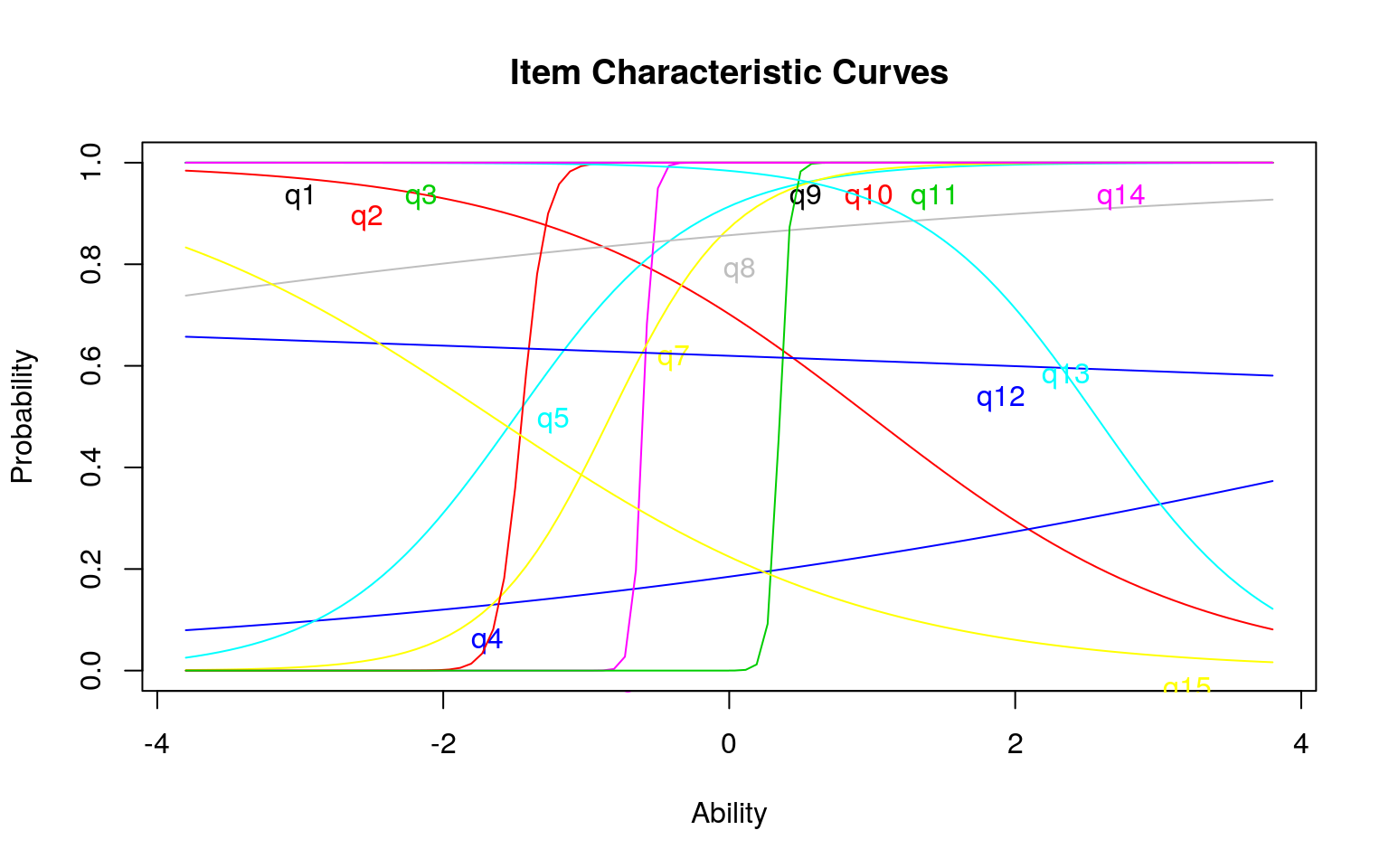model4a  Call: ltm(formula = dat[, 1:15] ~ z1) Coefficients: Dffclt Dscrmn q1 -1.802629e+08 0.000 q2 9.910000e-01 -0.864 q3 -1.802629e+08 0.000 q4 5.842000e+00 0.254 q5 -1.494000e+00 1.581 q6 -6.030000e-01 28.245 q7 -8.320000e-01 2.301 q8 -9.028000e+00 0.198 q9 -1.802629e+08 0.000 q10 -1.449000e+00 12.044 q11 3.520000e-01 27.475 q12 1.140900e+01 -0.043 q13 2.567000e+00 -1.604 q14 -1.802629e+08 0.000 q15 -1.656000e+00 -0.750 Log.Lik: -99.018 # summary(model4) item.fit(model4a)  Item-Fit Statistics and P-values Call: ltm(formula = dat[, 1:15] ~ z1) Alternative: Items do not fit the model Ability Categories: 10 X^2 Pr(>X^2) q1 0.0000 1 q2 15.4790 0.0505 q3 0.0000 1 q4 13.1159 0.1079 q5 9.8377 0.2766 q6 0.0956 1 q7 5.3522 0.7194 q8 8.1935 0.4148 q9 0.0000 1 q10 1.9348 0.9829 q11 0.0621 1 q12 13.6124 0.0924 q13 5.8759 0.6611 q14 0.0000 1 q15 6.9981 0.5368 model4b <- ltm(dat[, 1:15] ~ z1 + z2) model4b  Call: ltm(formula = dat[, 1:15] ~ z1 + z2) Coefficients: (Intercept) z1 z2 q1 65.566 0.000 0.000 q2 1.236 -0.537 1.942 q3 65.566 0.000 0.000 q4 -121.843 105.913 59.674 q5 185.353 107.015 -142.073 q6 47.280 98.106 -39.255 q7 103.551 147.976 37.214 q8 2.165 0.775 0.896 q9 65.566 0.000 0.000 q10 143.812 93.717 -18.639 q11 -74.365 111.343 -148.643 q12 0.523 0.274 0.552 q13 3.472 -0.503 0.906 q14 65.566 0.000 0.000 q15 -1.578 -0.019 1.505 Log.Lik: -85.347 anova(model4a, model4b)  Likelihood Ratio Table AIC BIC log.Lik LRT df p.value model4a 258.04 289.37 -99.02 model4b 260.69 307.70 -85.35 27.34 15 0.026 # item.fit(model4b) fs <- factor.scores(model4b) barplot(t(fs$coef), beside = T)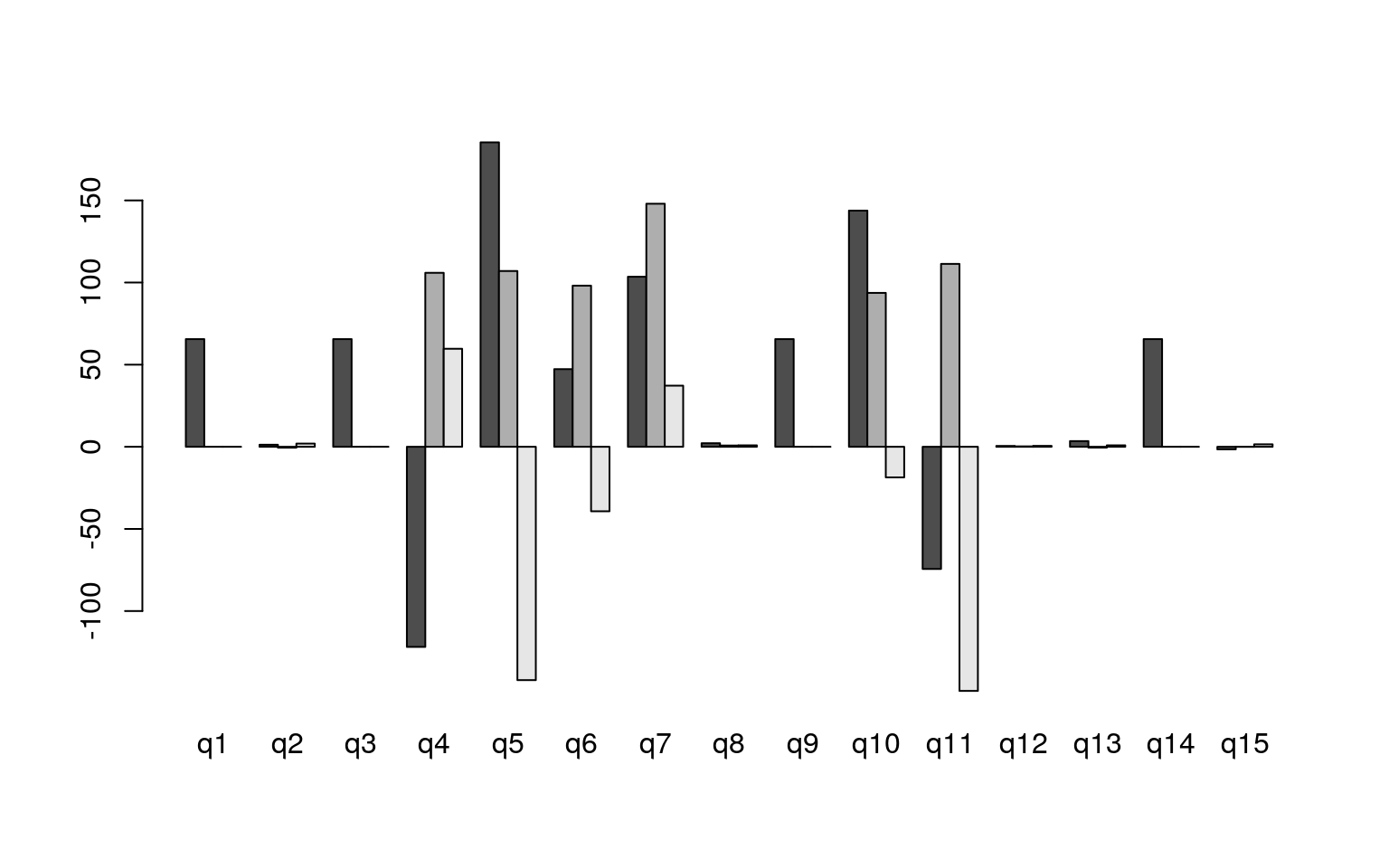plot(fs$coef[, 2], fs$coef[, 3])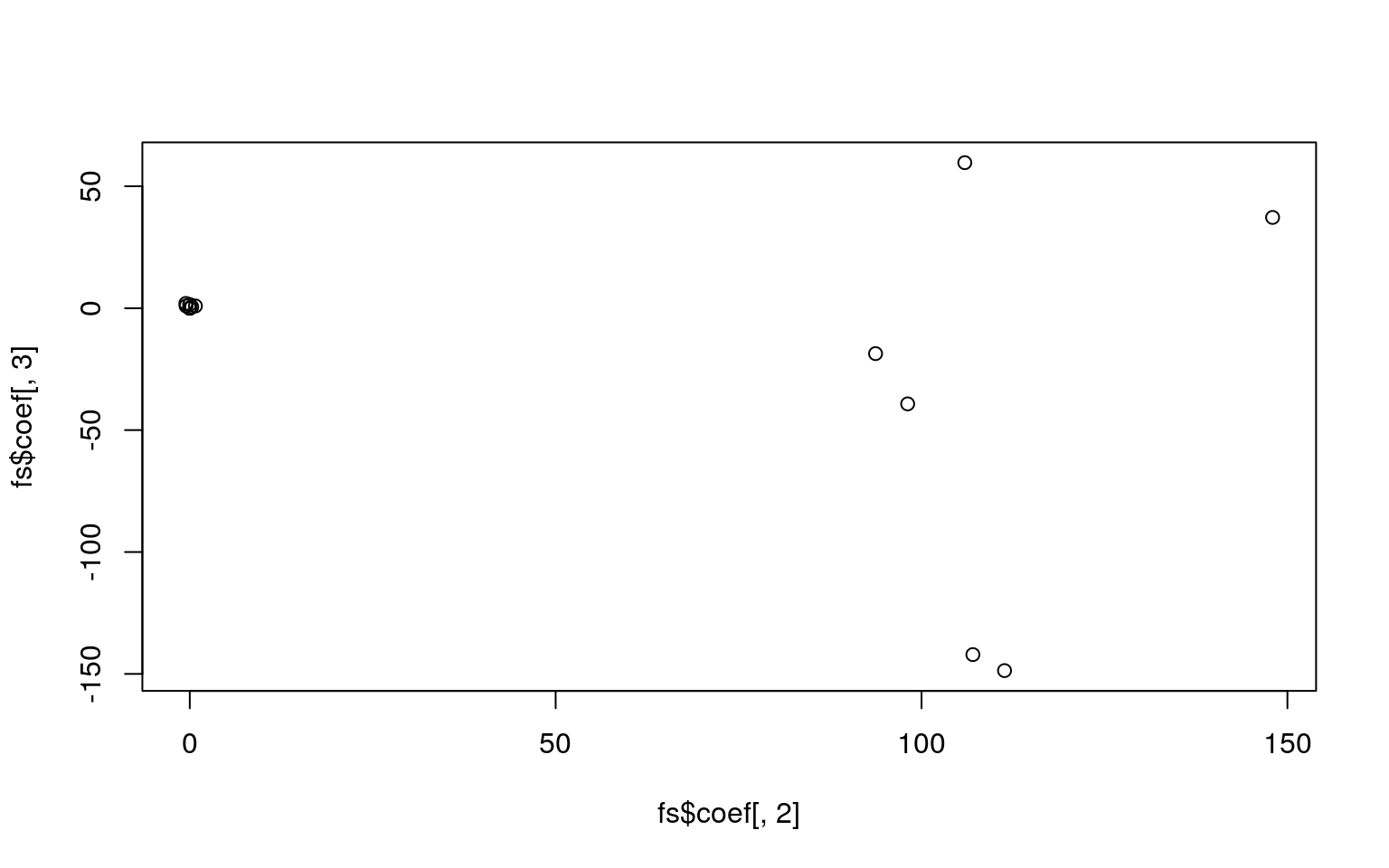## Guessing parameters: the three-parameter model

If you have questions that differ in the ability to get the question right by chance, you might want to incorporate a guessing parameter. These are just the normal ltm model, but bottom out at a lower level that you either estimate or specify. This might be useful if you have a true/false test, where accuracy should be at least 50%, especially if this is mixed with other questions like short answer or multiple choice where guessing accuracy would be lower. In this case, you could fix the parameters based on question type. Otherwise, you might want to estimate them directly–but you would need to be sure you had enough data to get good estimates.

This model is called the three-parameter model (TPM). It incorporates a guessing value, if the chance of getting an answer right is non-zero by guessing.

model9 <- tpm(dat[, 1:15], type = "latent.trait", max.guessing = 0.5)
model9

Call:
tpm(data = dat[, 1:15], type = "latent.trait", max.guessing = 0.5)

Coefficients:
Gussng         Dffclt   Dscrmn
q1    0.025  -4.775907e+08    0.000
q2    0.054  -9.160000e-01    0.806
q3    0.029  -4.775662e+08    0.000
q4    0.174  -1.884500e+01   -0.200
q5    0.113   1.031000e+00   -3.043
q6    0.014   4.510000e-01  -18.446
q7    0.463   1.230000e-01   -6.073
q8    0.051   2.353800e+01   -0.074
q9    0.032  -4.775418e+08    0.000
q10   0.048   1.513000e+00   -6.885
q11   0.000  -3.800000e-01  -22.118
q12   0.072  -2.603000e+00    0.144
q13   0.048  -1.916000e+00    3.428
q14   0.036  -4.775173e+08    0.000
q15   0.003   1.715000e+00    0.722

Log.Lik: -98.513
plot(model9)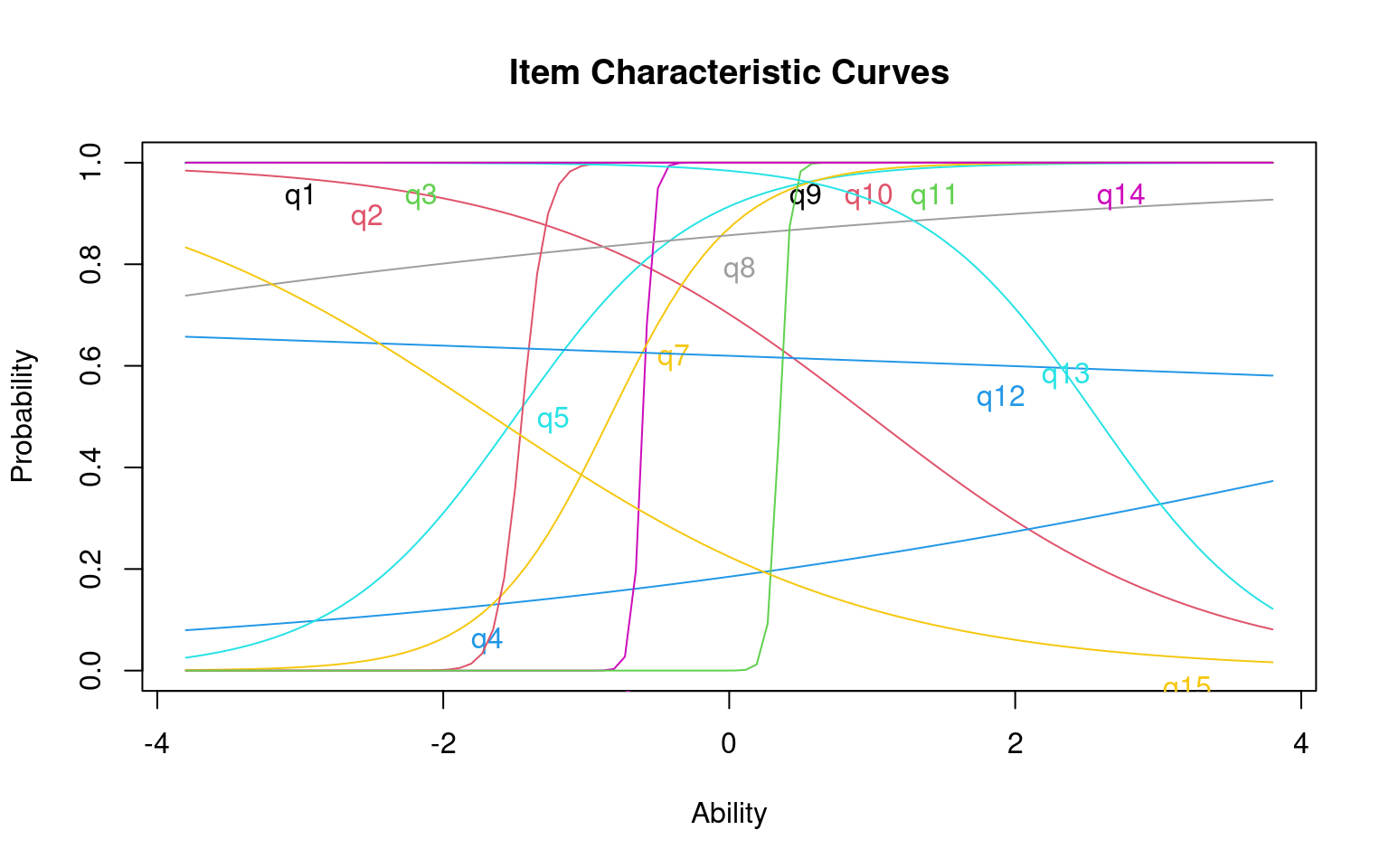Notice how different items bottom out at different levels.

With a small class, there are a lot of items with negative discriminability. Let’s look at how they work out, by comparing average test score to particular answers:

par(mfrow = c(2, 2))
boxplot(dat$q5, rowMeans(dat), main = "Correct on q5", names = c("Incorrect (3)", "correct (18)")) boxplot(dat$q4, rowMeans(dat), main = "Correct on q4", names = c("Incorrect (1)",
"correct (20)"))

boxplot(dat$q10, rowMeans(dat), main = "Correct on q10", names = c("Incorrect (3)", "correct (18)")) boxplot(dat$q11, rowMeans(dat), main = "Correct on q11", names = c("Incorrect (13)",
"correct (8)"))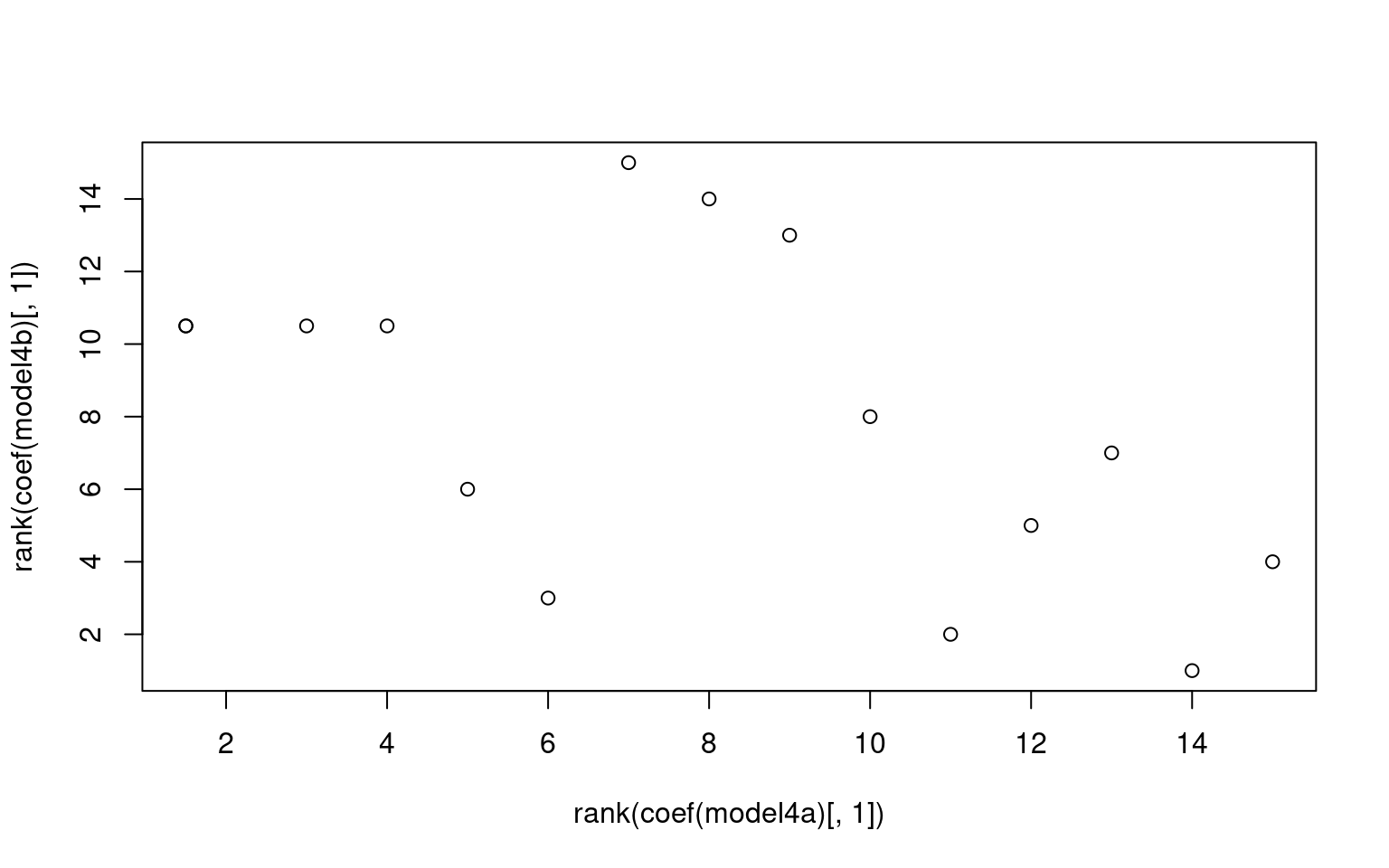We can see that for some of these, accuracy on the question is negatively correlated with accuracy on the test. For others, there are other strange things, like very small numbers of errors that might make estimation difficult.

# Information curves

Each questions can be transformed into an information score, which is the distribution of information implied by the cumulative score. Also, you can plot the characteristic of the entire test:

plot(model4a)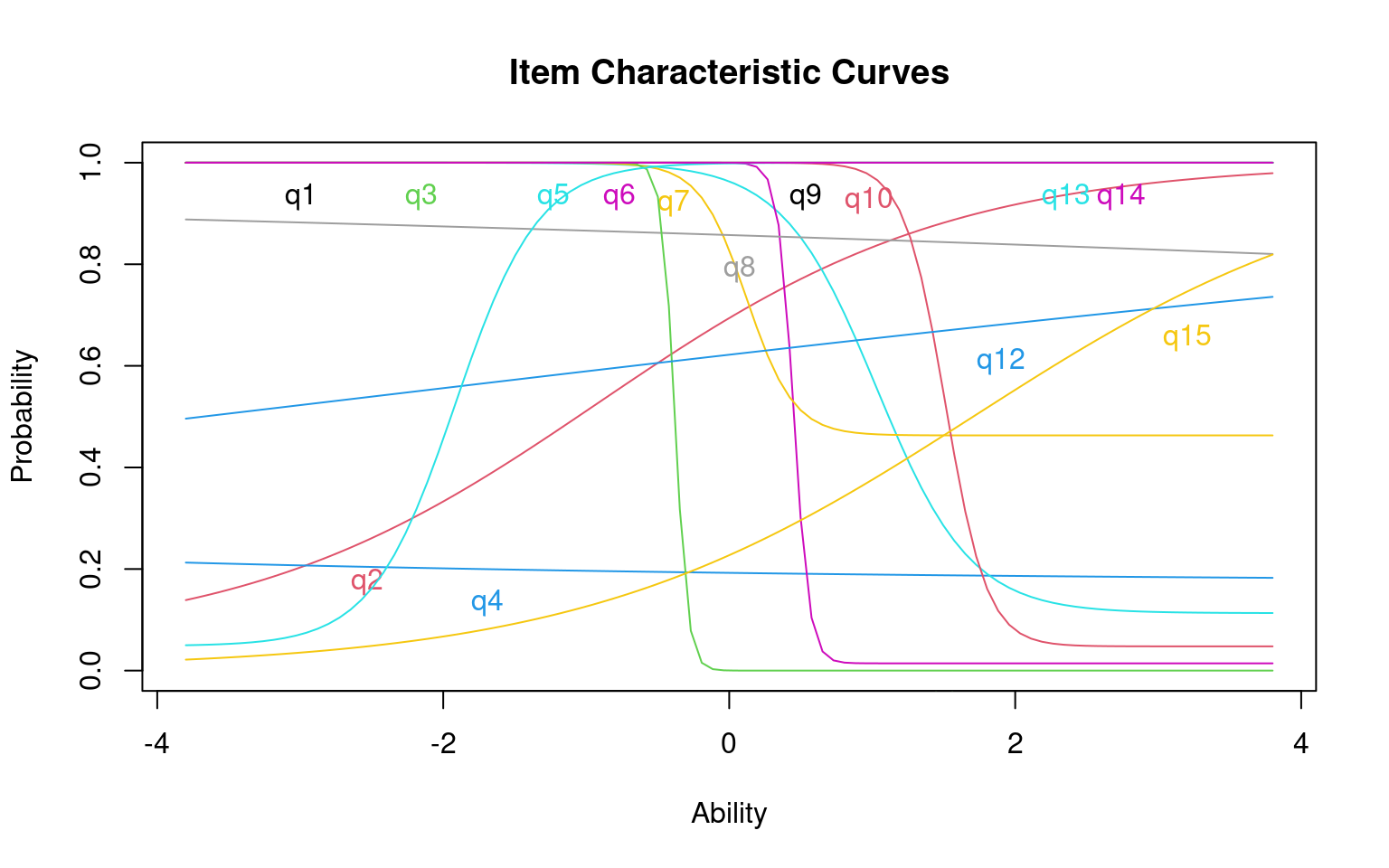plot(model4a, legend = T, type = "IIC", items = 1:5)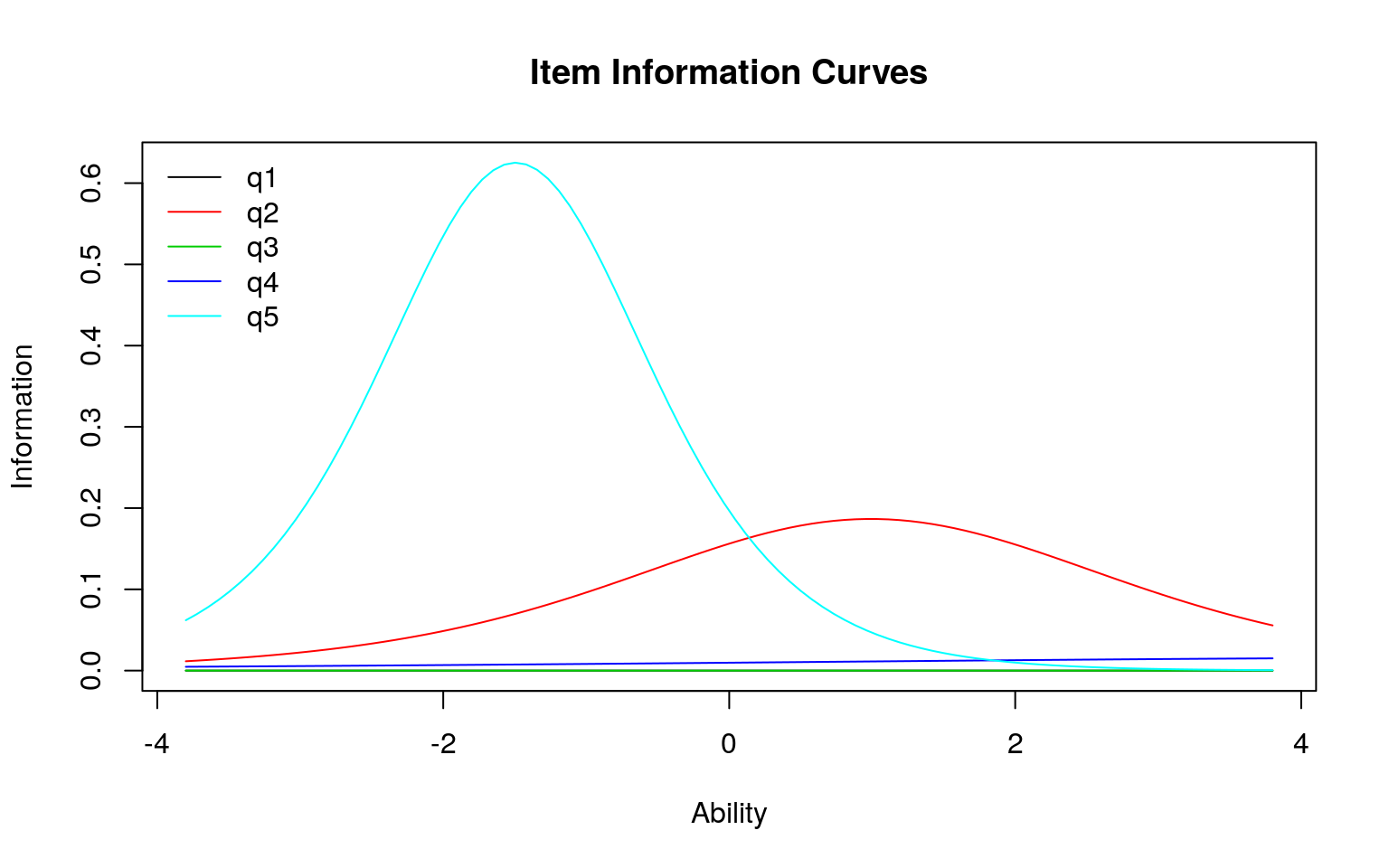plot(model4a, type = "IIC", legend = T, item = c(1:15)[c(-11, -6, -10)])The height of the curve indicates where the most informative ability level for each question is. A very discriminative question will have a sharp rise at a specific point, and you would be good at separating those below from those above.

model5 <- ltm(dat[, c(1, 3, 5, 9, 14)] ~ z1)
model5

Call:
ltm(formula = dat[, c(1, 3, 5, 9, 14)] ~ z1)

Coefficients:
Dffclt  Dscrmn
q1   -1.321958e+11   0.000
q3   -1.321958e+11   0.000
q5    1.188000e+00  -3.667
q9   -1.321958e+11   0.000
q14  -1.321958e+11   0.000

Log.Lik: -8.612
plot(model5, legend = T, type = "IIC")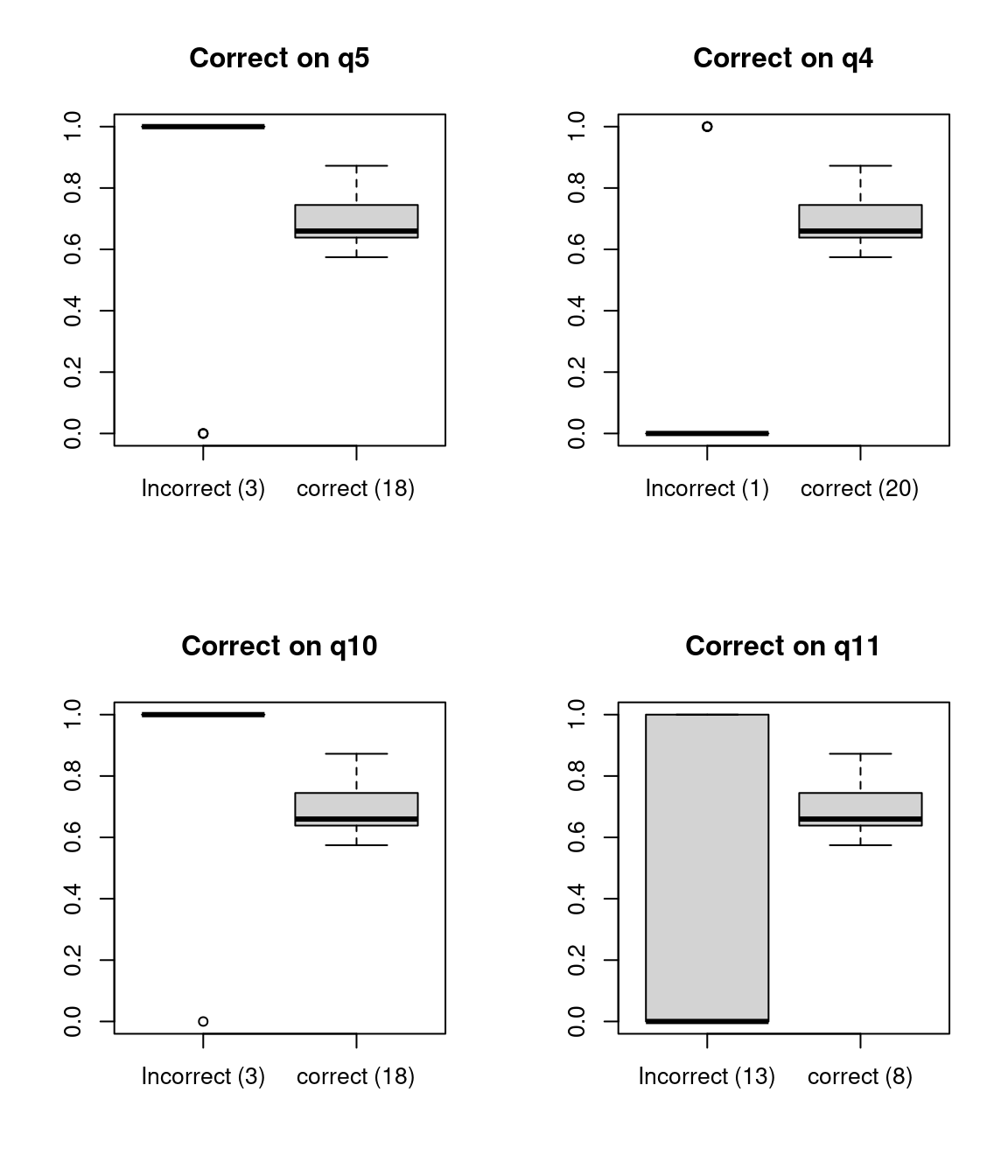You can specify different items, or items=0 tells you the entire test. This tells you the range of abilities that the test or items will be good at. You can also specify a range to integrate over, to see which range the test is best at discriminating. This can be used to understand whether the test is good at discrimating low-performers (maybe a test for remidial instruction) on high-performers (a test for entrance into a competitive class or program).

plot(model5, legend = T, type = "IIC", items = 0)info <- information(model5, c(-4, 4))
info

Call:
ltm(formula = dat[, c(1, 3, 5, 9, 14)] ~ z1)

Total Information = 3.67
Information in (-4, 4) = 3.67 (100%)
Based on all the items

# Graded response model and partial credit model.

The basic assumptions of IRT is that you have a binary outcome (correct or incorrect). But it could be interesting to do an IRT-like analysis for non-binary responses. If you have a set of likert-scale responses, where they are all coded in the same direction, and they each independently give support for some construct, you can use a graded response model. This might be useful for personality data, for example. Let’s consider measures from the big five personality questionnare we have examined in the past.

A related model in the ltm package is the graded partial credit model (gpcm). This would allow you to place an ordinal scale on correctness, and do an IRT analysis. Maybe in a short answer response, you score full credit for one response, and partial credit for another. We won’t cover this model here, but it has some similarity to the GRM.

To examine the GRM, Let’s obtain just the introversion/extraversion values, and reverse code so they are all in the same direction. for convenience, I’ll also remove any values that are NA.

big5 <- read.csv("bigfive.csv")
qtype <- c("E", "A", "C", "N", "O", "E", "A", "C", "N", "O", "E", "A", "C",
"N", "O", "E", "A", "C", "N", "O", "E", "A", "C", "N", "O", "E", "A", "C",
"N", "O", "E", "A", "C", "N", "O", "E", "A", "C", "N", "O", "O", "A", "C",
"O")
valence <- c(1, -1, 1, 1, 1, -1, 1, -1, -1, 1, 1, -1, 1, 1, 1, 1, 1, -1, 1,
1, -1, 1, -1, -1, 1, 1, -1, 1, 1, 1, -1, 1, 1, -1, -1, 1, -1, 1, 1, 1, -1,
1, -1, 1)
## reverse code
for (i in 2:ncol(big5)) {
if (valence[i - 1] == -1) {
big5[, i] <- 6 - big5[, i]
}
}
ei <- big5[, c(T, qtype == "E")]
ei <- ei[!is.na(rowSums(ei)), ]

Now, the graded response model in ltm (grm) will do a irt-like analysis, treating these as ordinal values. You can use a constrained or unconstrained model–the constrained model fits an equal discriminability across all questions. Because we have a lot of data, this model takes a while to fit.

g1 <- grm(ei[, -1], constrained = TRUE)
g1

Call:
grm(data = ei[, -1], constrained = TRUE)

Coefficients:
Extrmt1  Extrmt2  Extrmt3  Extrmt4  Dscrmn
Q1    -2.210   -0.924   -0.150    1.233   1.684
Q6    -1.531    0.123    0.838    2.007   1.684
Q11   -2.729   -1.194   -0.359    1.068   1.684
Q16   -2.853   -1.410   -0.370    1.061   1.684
Q21   -1.356    0.100    0.702    1.863   1.684
Q26   -2.003   -0.861   -0.116    1.360   1.684
Q31   -1.389    0.368    0.865    1.971   1.684
Q36   -2.579   -1.099   -0.468    0.984   1.684

Log.Lik: -10583.71
summary(g1)

Call:
grm(data = ei[, -1], constrained = TRUE)

Model Summary:
log.Lik      AIC     BIC
-10583.71 21233.41 21395.7

Coefficients:
$Q1 value Extrmt1 -2.210 Extrmt2 -0.924 Extrmt3 -0.150 Extrmt4 1.233 Dscrmn 1.684$Q6
value
Extrmt1 -1.531
Extrmt2  0.123
Extrmt3  0.838
Extrmt4  2.007
Dscrmn   1.684

$Q11 value Extrmt1 -2.729 Extrmt2 -1.194 Extrmt3 -0.359 Extrmt4 1.068 Dscrmn 1.684$Q16
value
Extrmt1 -2.853
Extrmt2 -1.410
Extrmt3 -0.370
Extrmt4  1.061
Dscrmn   1.684

$Q21 value Extrmt1 -1.356 Extrmt2 0.100 Extrmt3 0.702 Extrmt4 1.863 Dscrmn 1.684$Q26
value
Extrmt1 -2.003
Extrmt2 -0.861
Extrmt3 -0.116
Extrmt4  1.360
Dscrmn   1.684

$Q31 value Extrmt1 -1.389 Extrmt2 0.368 Extrmt3 0.865 Extrmt4 1.971 Dscrmn 1.684$Q36
value
Extrmt1 -2.579
Extrmt2 -1.099
Extrmt3 -0.468
Extrmt4  0.984
Dscrmn   1.684

Integration:
method: Gauss-Hermite

Optimization:
Convergence: 0
quasi-Newton: BFGS 

We can see that each question is modeled with its own IRT-like model. There are five levels here, and four transitions between levels, which are modeled as sort of difficulty parameters for each transition between items.

Plotting each question gives us another look

par(mfrow = c(4, 2))
plot(g1, items = 1)
plot(g1, items = 2)
plot(g1, items = 3)
plot(g1, items = 4)
plot(g1, items = 5)
plot(g1, items = 6)
plot(g1, items = 7)
plot(g1, items = 8)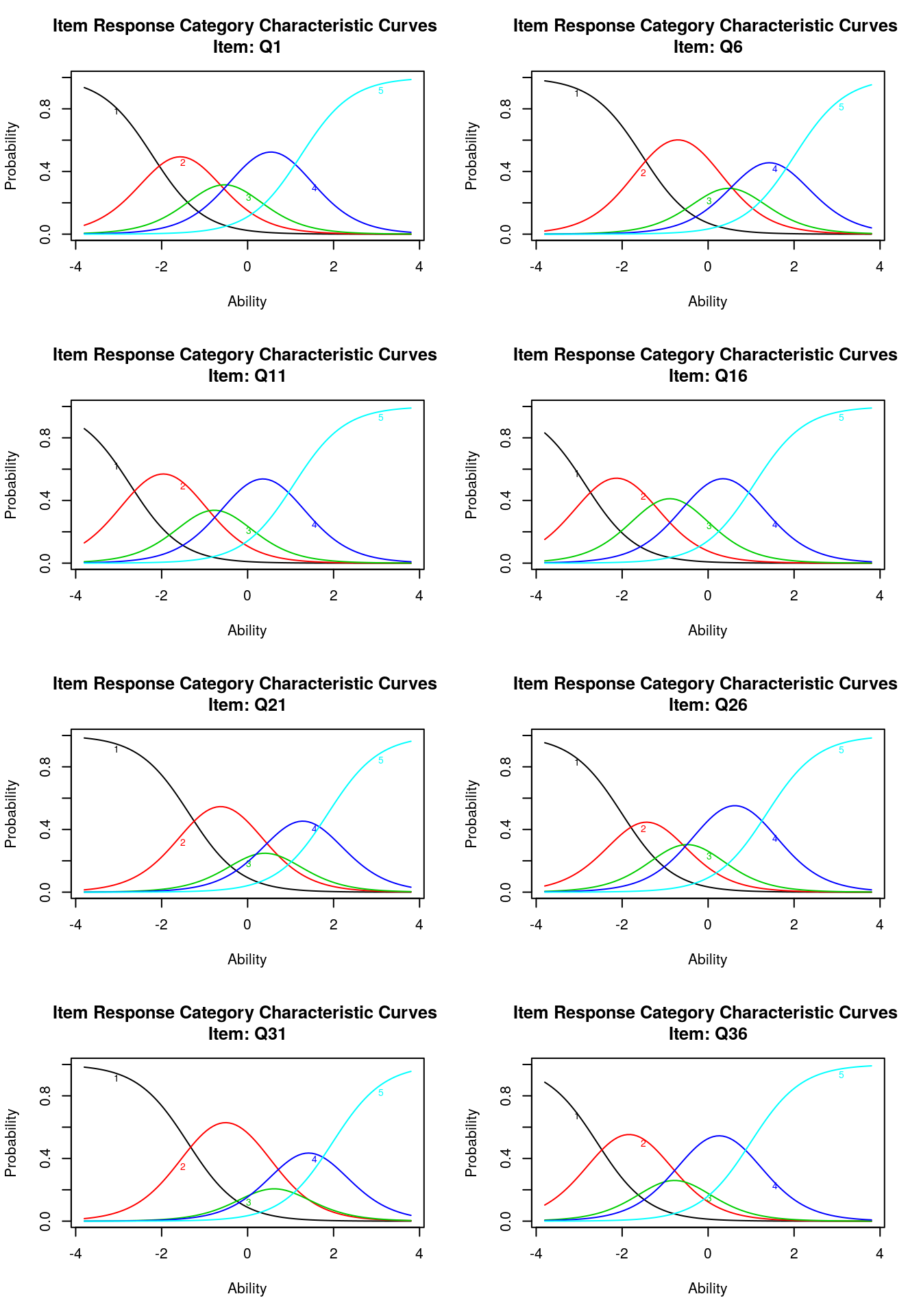The margins() function works here as well. We can see that there are a couple that violate the two-way independence (q1-q21; q6-q21, etc.)

margins(g1)

Call:
grm(data = ei[, -1], constrained = TRUE)

Fit on the Two-Way Margins

Q1  Q6     Q11    Q16    Q21    Q26    Q31    Q36
Q1  -    25.82  50.38  37.85 102.57  67.00  82.91  72.89
Q6      -       44.89  50.86 124.08  73.31  44.03  37.03
Q11            -       96.73  55.00  76.17  84.33  45.93
Q16            ***    -       55.38  51.31  65.46  52.74
Q21 *** ***                  -       53.24  74.63  29.73
Q26                                 -       62.84  37.69
Q31                                        -       34.28
Q36                                               -

'***' denotes pairs of items with lack-of-fit

Let’s fit this unconstrained:

g2 <- grm(ei[, -1], constrained = FALSE)
g2

Call:
grm(data = ei[, -1], constrained = FALSE)

Coefficients:
Extrmt1  Extrmt2  Extrmt3  Extrmt4  Dscrmn
Q1    -1.927   -0.814   -0.137    1.076   2.215
Q6    -1.531    0.121    0.838    2.010   1.682
Q11   -2.916   -1.276   -0.384    1.140   1.509
Q16   -2.766   -1.370   -0.363    1.029   1.773
Q21   -1.269    0.090    0.655    1.742   1.907
Q26   -2.549   -1.088   -0.137    1.732   1.164
Q31   -1.511    0.395    0.939    2.150   1.459
Q36   -2.302   -0.988   -0.426    0.877   2.090

Log.Lik: -10539.91
summary(g2)

Call:
grm(data = ei[, -1], constrained = FALSE)

Model Summary:
log.Lik      AIC      BIC
-10539.91 21159.82 21356.53

Coefficients:
$Q1 value Extrmt1 -1.927 Extrmt2 -0.814 Extrmt3 -0.137 Extrmt4 1.076 Dscrmn 2.215$Q6
value
Extrmt1 -1.531
Extrmt2  0.121
Extrmt3  0.838
Extrmt4  2.010
Dscrmn   1.682

$Q11 value Extrmt1 -2.916 Extrmt2 -1.276 Extrmt3 -0.384 Extrmt4 1.140 Dscrmn 1.509$Q16
value
Extrmt1 -2.766
Extrmt2 -1.370
Extrmt3 -0.363
Extrmt4  1.029
Dscrmn   1.773

$Q21 value Extrmt1 -1.269 Extrmt2 0.090 Extrmt3 0.655 Extrmt4 1.742 Dscrmn 1.907$Q26
value
Extrmt1 -2.549
Extrmt2 -1.088
Extrmt3 -0.137
Extrmt4  1.732
Dscrmn   1.164

$Q31 value Extrmt1 -1.511 Extrmt2 0.395 Extrmt3 0.939 Extrmt4 2.150 Dscrmn 1.459$Q36
value
Extrmt1 -2.302
Extrmt2 -0.988
Extrmt3 -0.426
Extrmt4  0.877
Dscrmn   2.090

Integration:
method: Gauss-Hermite

Optimization:
Convergence: 0
quasi-Newton: BFGS 
par(mfrow = c(4, 2))
plot(g2, items = 1)
plot(g2, items = 2)
plot(g2, items = 3)
plot(g2, items = 4)
plot(g2, items = 5)
plot(g2, items = 6)
plot(g2, items = 7)
plot(g2, items = 8)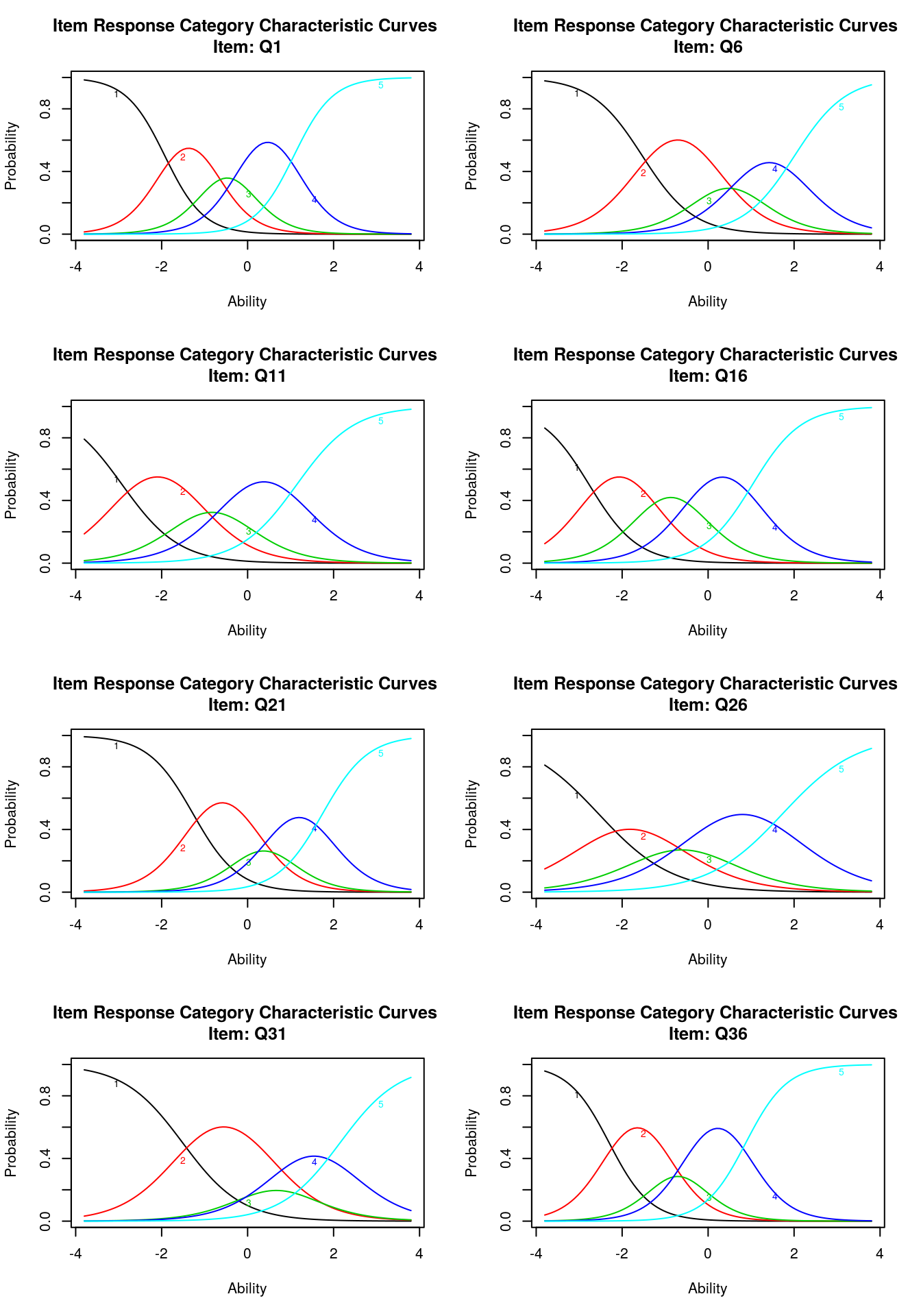For this model, we might consider the midpoint transition (extrm2) s th ‘center’ of the question. We can see that Q36 and Q26 are low, while Q21 and Q31 are high. We might also use this to infer that a 4 on Q36 is about equivalent to a 3 on Q21.

2019-02-28